Скачать презентацию Spatial Statistics and Spatial Knowledge Discovery First law

5a2b319f81973f4faebcc4003085b815.ppt

• Количество слайдов: 82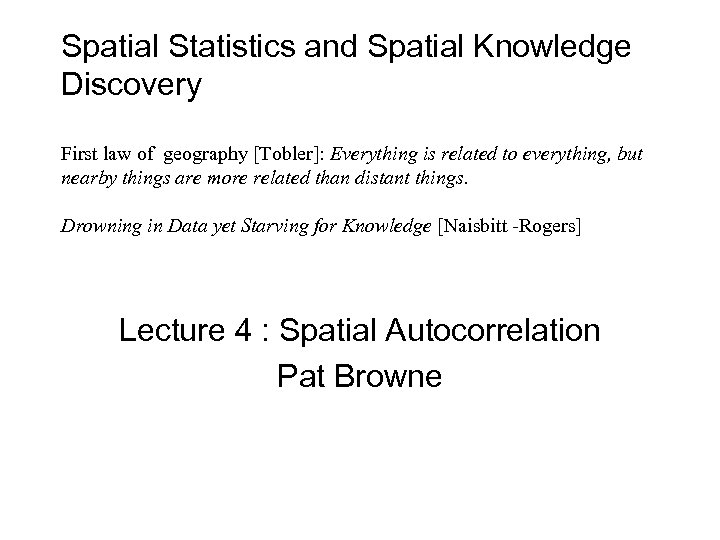Spatial Statistics and Spatial Knowledge Discovery First law of geography [Tobler]: Everything is related to everything, but nearby things are more related than distant things. Drowning in Data yet Starving for Knowledge [Naisbitt -Rogers] Lecture 4 : Spatial Autocorrelation Pat Browne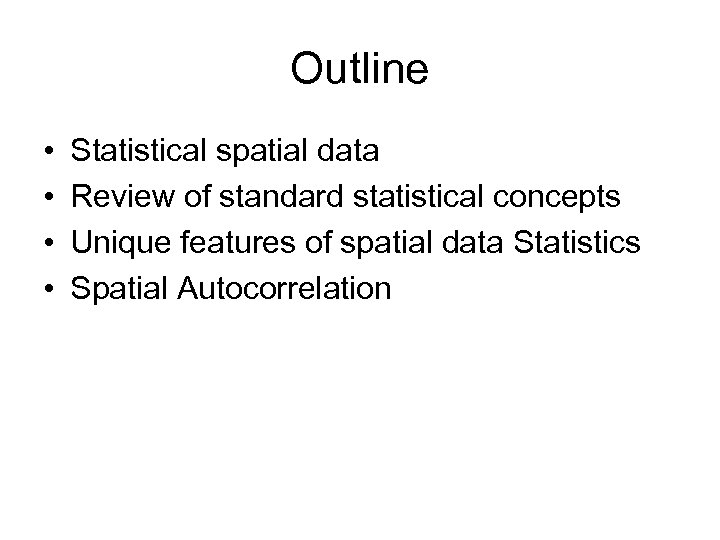Outline • • Statistical spatial data Review of standard statistical concepts Unique features of spatial data Statistics Spatial Autocorrelation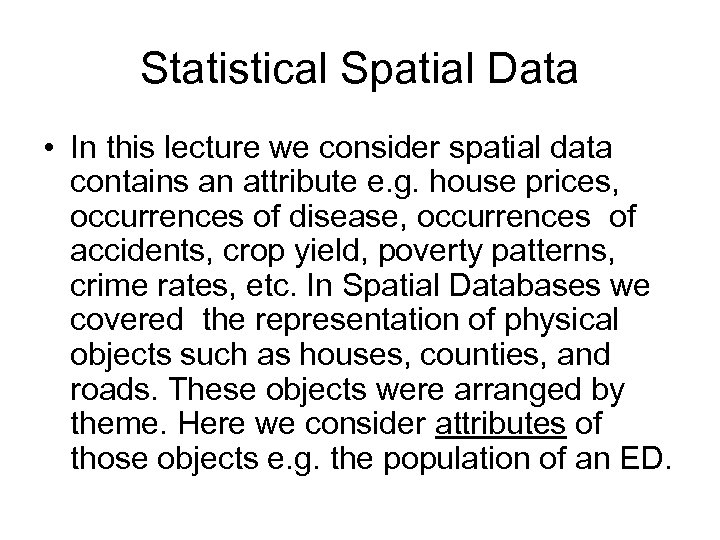Statistical Spatial Data • In this lecture we consider spatial data contains an attribute e. g. house prices, occurrences of disease, occurrences of accidents, crop yield, poverty patterns, crime rates, etc. In Spatial Databases we covered the representation of physical objects such as houses, counties, and roads. These objects were arranged by theme. Here we consider attributes of those objects e. g. the population of an ED.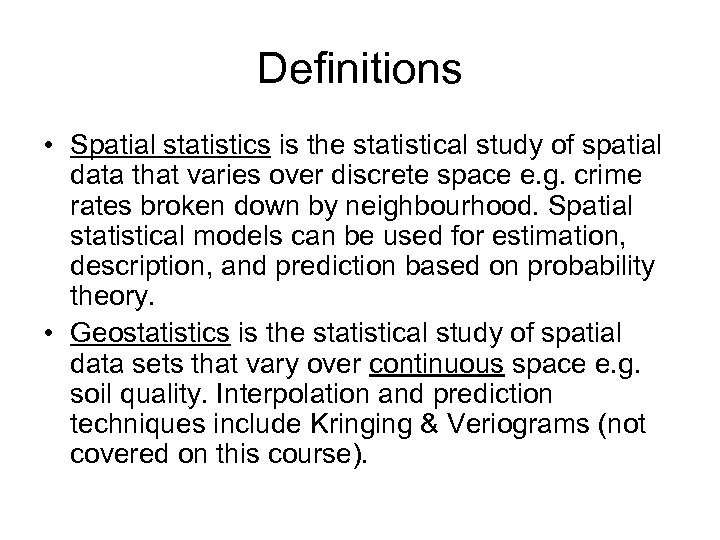Definitions • Spatial statistics is the statistical study of spatial data that varies over discrete space e. g. crime rates broken down by neighbourhood. Spatial statistical models can be used for estimation, description, and prediction based on probability theory. • Geostatistics is the statistical study of spatial data sets that vary over continuous space e. g. soil quality. Interpolation and prediction techniques include Kringing & Veriograms (not covered on this course).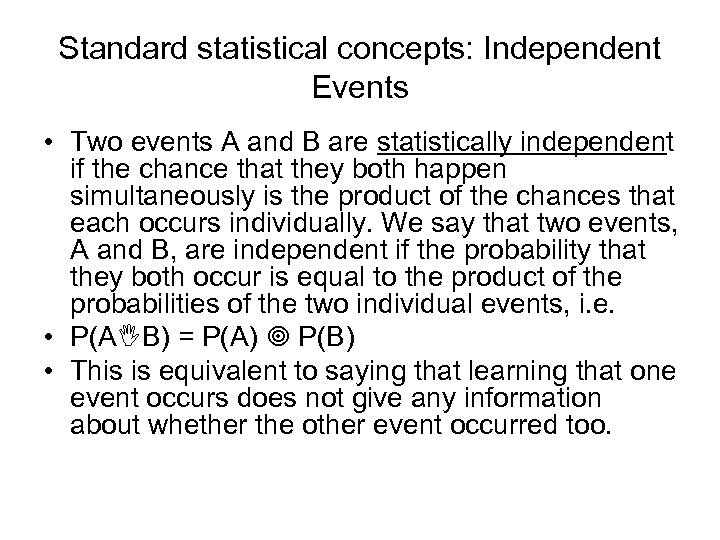Standard statistical concepts: Independent Events • Two events A and B are statistically independent if the chance that they both happen simultaneously is the product of the chances that each occurs individually. We say that two events, A and B, are independent if the probability that they both occur is equal to the product of the probabilities of the two individual events, i. e. • P(A B) = P(A) P(B) • This is equivalent to saying that learning that one event occurs does not give any information about whether the other event occurred too.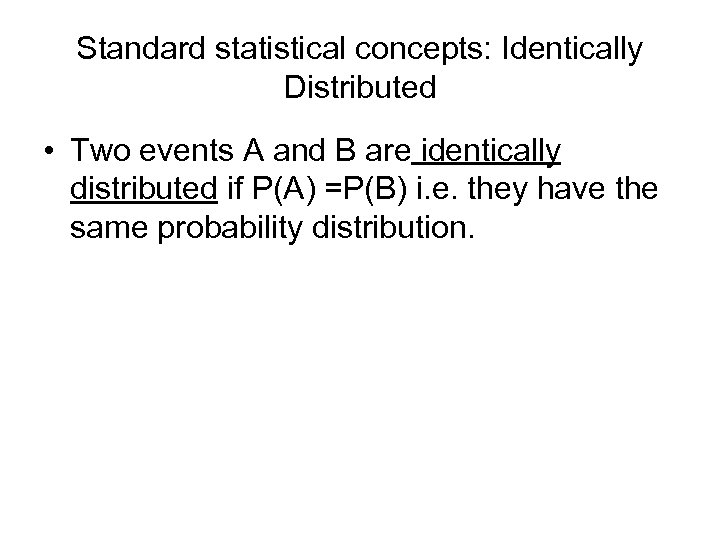Standard statistical concepts: Identically Distributed • Two events A and B are identically distributed if P(A) =P(B) i. e. they have the same probability distribution.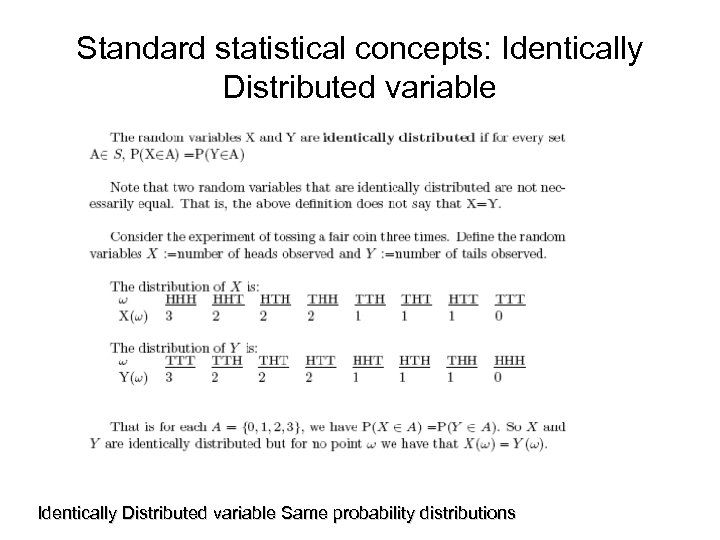Standard statistical concepts: Identically Distributed variable Same probability distributions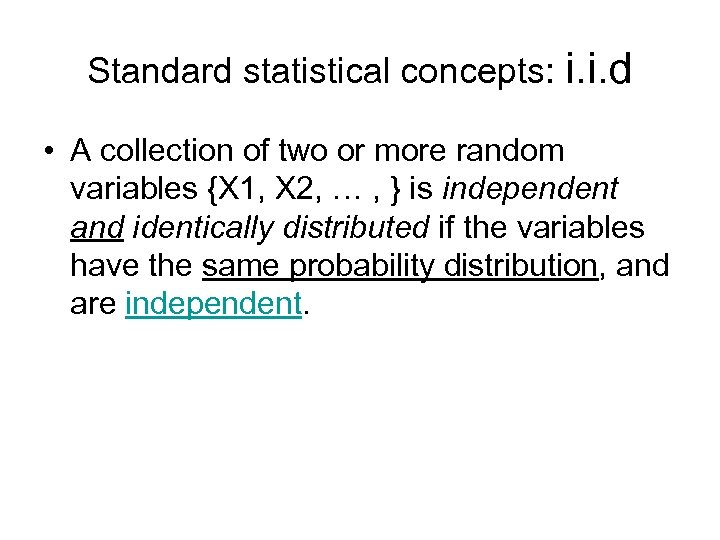Standard statistical concepts: i. i. d • A collection of two or more random variables {X 1, X 2, … , } is independent and identically distributed if the variables have the same probability distribution, and are independent.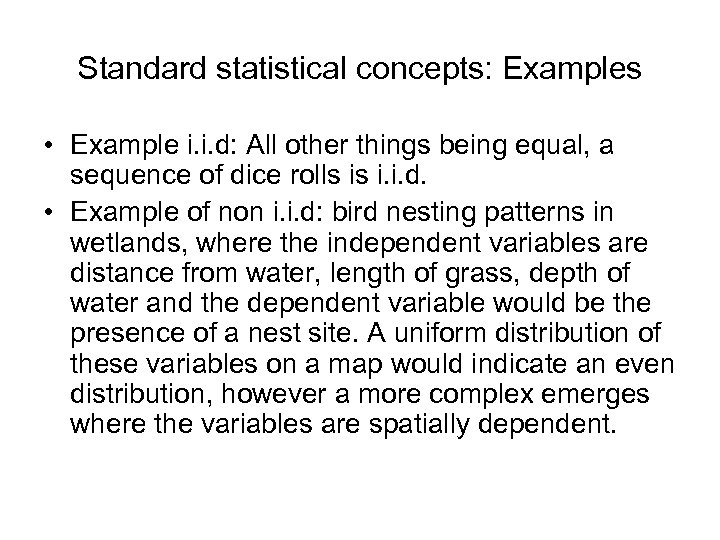Standard statistical concepts: Examples • Example i. i. d: All other things being equal, a sequence of dice rolls is i. i. d. • Example of non i. i. d: bird nesting patterns in wetlands, where the independent variables are distance from water, length of grass, depth of water and the dependent variable would be the presence of a nest site. A uniform distribution of these variables on a map would indicate an even distribution, however a more complex emerges where the variables are spatially dependent.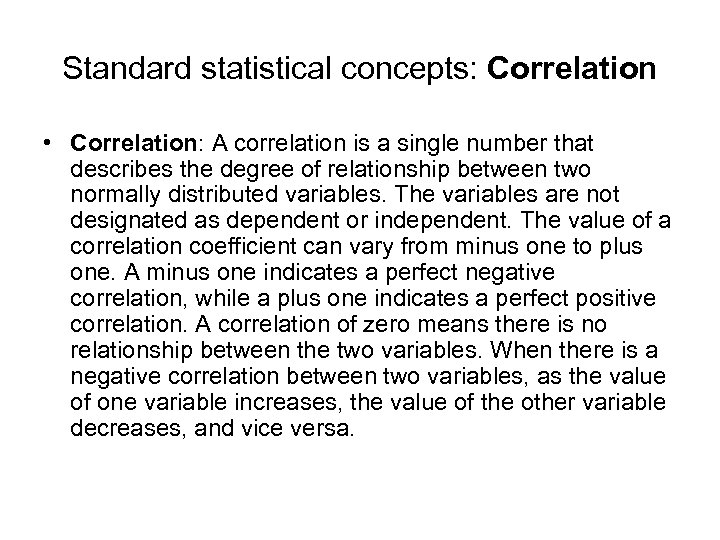Standard statistical concepts: Correlation • Correlation: A correlation is a single number that describes the degree of relationship between two normally distributed variables. The variables are not designated as dependent or independent. The value of a correlation coefficient can vary from minus one to plus one. A minus one indicates a perfect negative correlation, while a plus one indicates a perfect positive correlation. A correlation of zero means there is no relationship between the two variables. When there is a negative correlation between two variables, as the value of one variable increases, the value of the other variable decreases, and vice versa.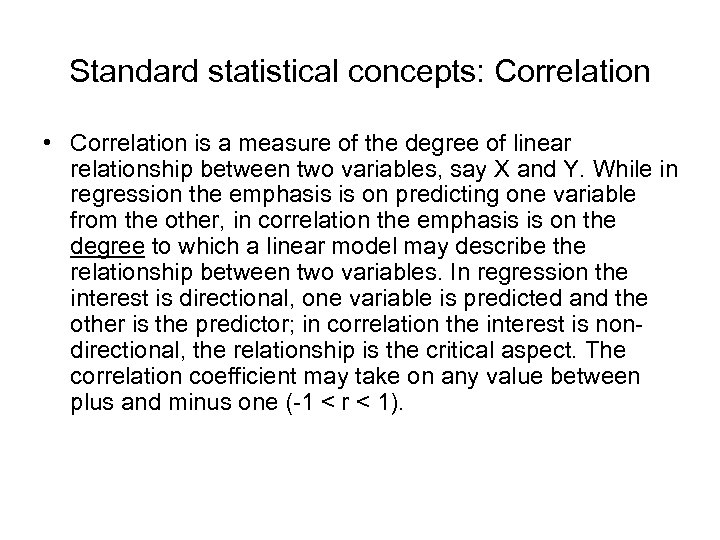Standard statistical concepts: Correlation • Correlation is a measure of the degree of linear relationship between two variables, say X and Y. While in regression the emphasis is on predicting one variable from the other, in correlation the emphasis is on the degree to which a linear model may describe the relationship between two variables. In regression the interest is directional, one variable is predicted and the other is the predictor; in correlation the interest is nondirectional, the relationship is the critical aspect. The correlation coefficient may take on any value between plus and minus one (-1 < r < 1).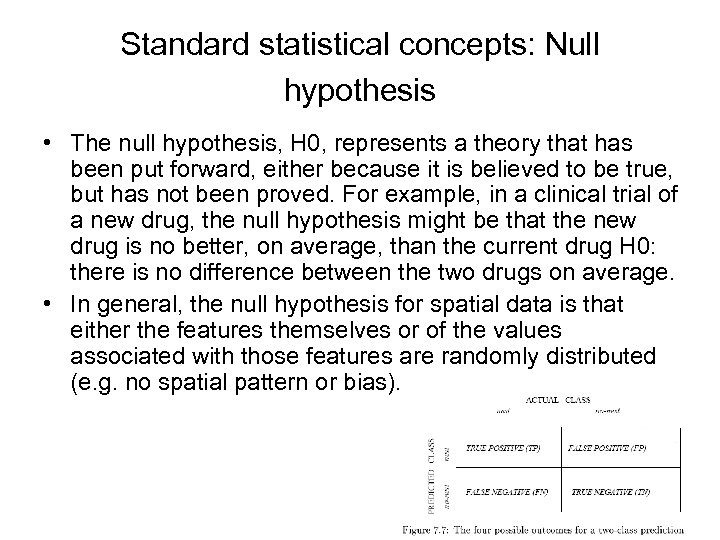Standard statistical concepts: Null hypothesis • The null hypothesis, H 0, represents a theory that has been put forward, either because it is believed to be true, but has not been proved. For example, in a clinical trial of a new drug, the null hypothesis might be that the new drug is no better, on average, than the current drug H 0: there is no difference between the two drugs on average. • In general, the null hypothesis for spatial data is that either the features themselves or of the values associated with those features are randomly distributed (e. g. no spatial pattern or bias).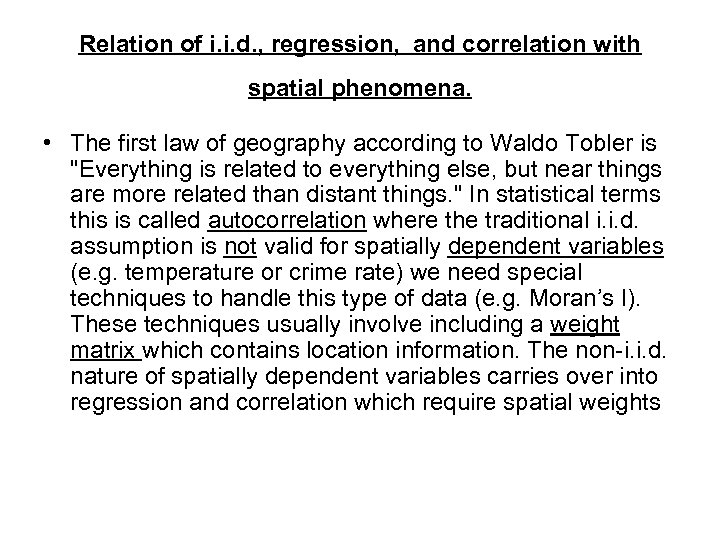Relation of i. i. d. , regression, and correlation with spatial phenomena. • The first law of geography according to Waldo Tobler is "Everything is related to everything else, but near things are more related than distant things. " In statistical terms this is called autocorrelation where the traditional i. i. d. assumption is not valid for spatially dependent variables (e. g. temperature or crime rate) we need special techniques to handle this type of data (e. g. Moran’s I). These techniques usually involve including a weight matrix which contains location information. The non-i. i. d. nature of spatially dependent variables carries over into regression and correlation which require spatial weights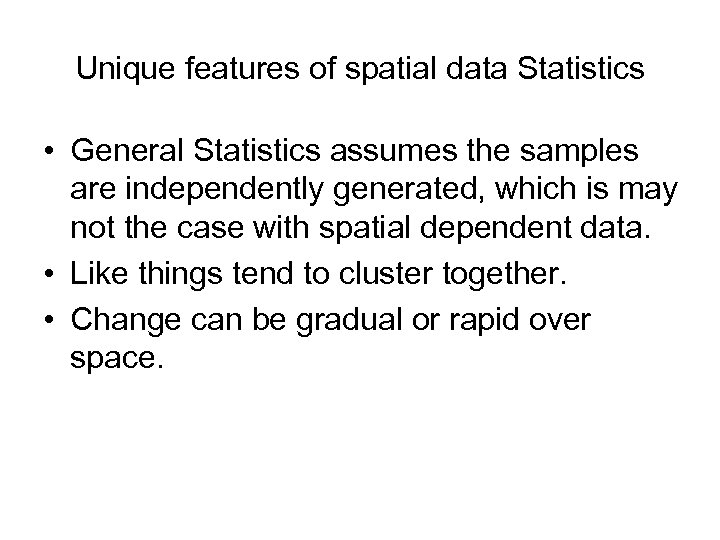Unique features of spatial data Statistics • General Statistics assumes the samples are independently generated, which is may not the case with spatial dependent data. • Like things tend to cluster together. • Change can be gradual or rapid over space.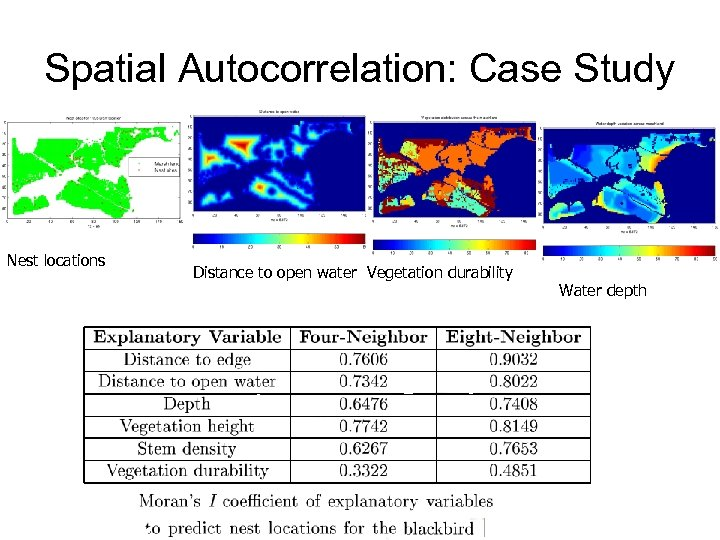Spatial Autocorrelation: Case Study Nest locations Distance to open water Vegetation durability Water depth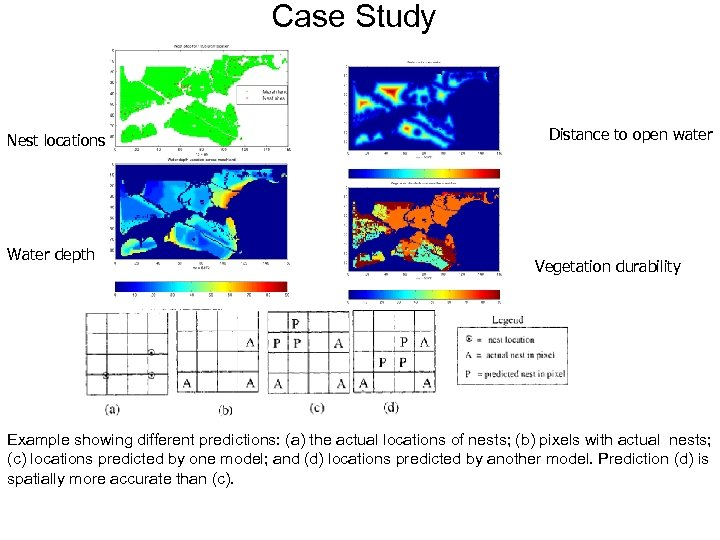Case Study Nest locations Water depth Distance to open water Vegetation durability Example showing different predictions: (a) the actual locations of nests; (b) pixels with actual nests; (c) locations predicted by one model; and (d) locations predicted by another model. Prediction (d) is spatially more accurate than (c).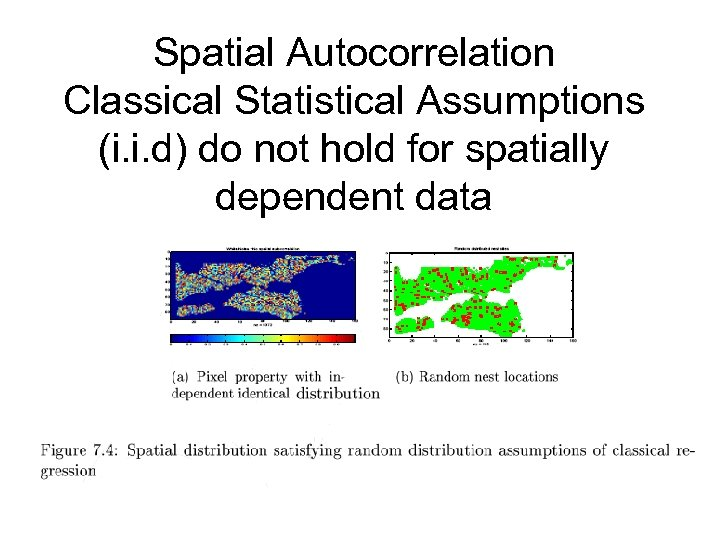Spatial Autocorrelation Classical Statistical Assumptions (i. i. d) do not hold for spatially dependent data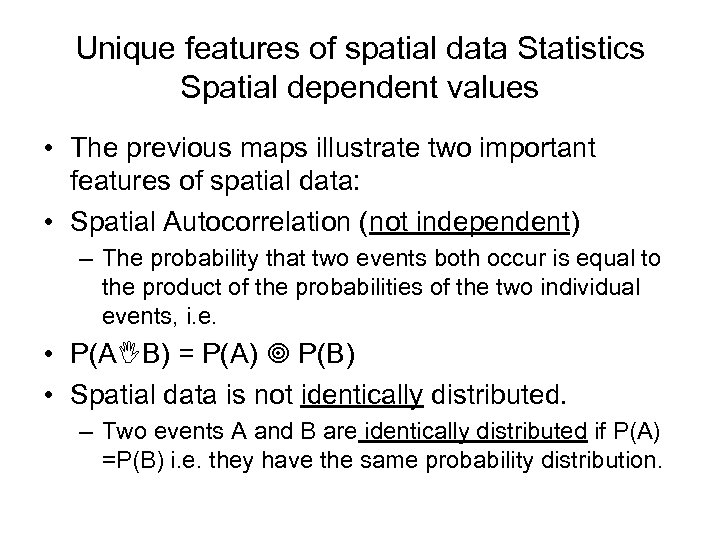Unique features of spatial data Statistics Spatial dependent values • The previous maps illustrate two important features of spatial data: • Spatial Autocorrelation (not independent) – The probability that two events both occur is equal to the product of the probabilities of the two individual events, i. e. • P(A B) = P(A) P(B) • Spatial data is not identically distributed. – Two events A and B are identically distributed if P(A) =P(B) i. e. they have the same probability distribution.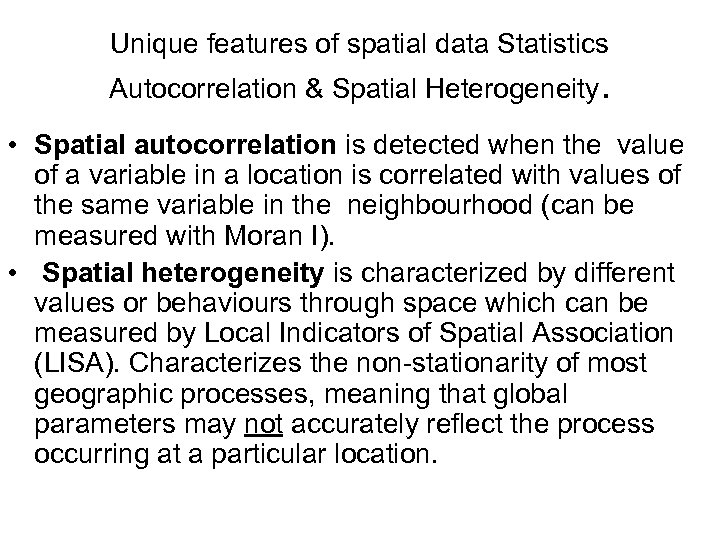Unique features of spatial data Statistics Autocorrelation & Spatial Heterogeneity. • Spatial autocorrelation is detected when the value of a variable in a location is correlated with values of the same variable in the neighbourhood (can be measured with Moran I). • Spatial heterogeneity is characterized by different values or behaviours through space which can be measured by Local Indicators of Spatial Association (LISA). Characterizes the non-stationarity of most geographic processes, meaning that global parameters may not accurately reflect the process occurring at a particular location.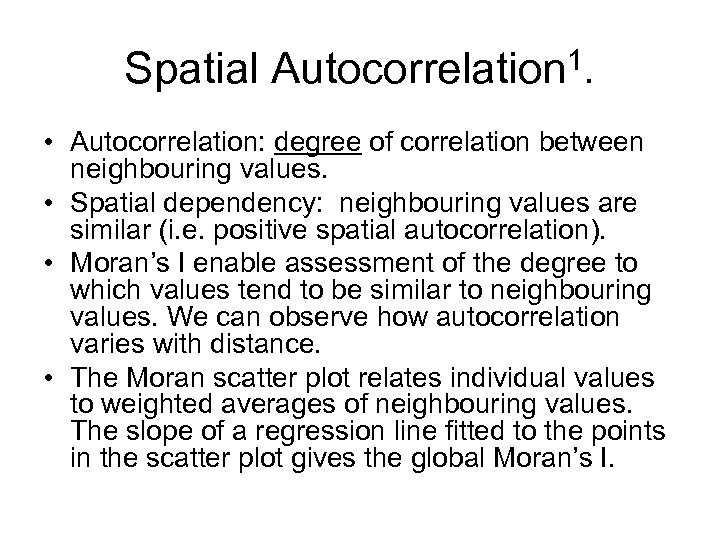Spatial Autocorrelation 1. • Autocorrelation: degree of correlation between neighbouring values. • Spatial dependency: neighbouring values are similar (i. e. positive spatial autocorrelation). • Moran’s I enable assessment of the degree to which values tend to be similar to neighbouring values. We can observe how autocorrelation varies with distance. • The Moran scatter plot relates individual values to weighted averages of neighbouring values. The slope of a regression line fitted to the points in the scatter plot gives the global Moran’s I.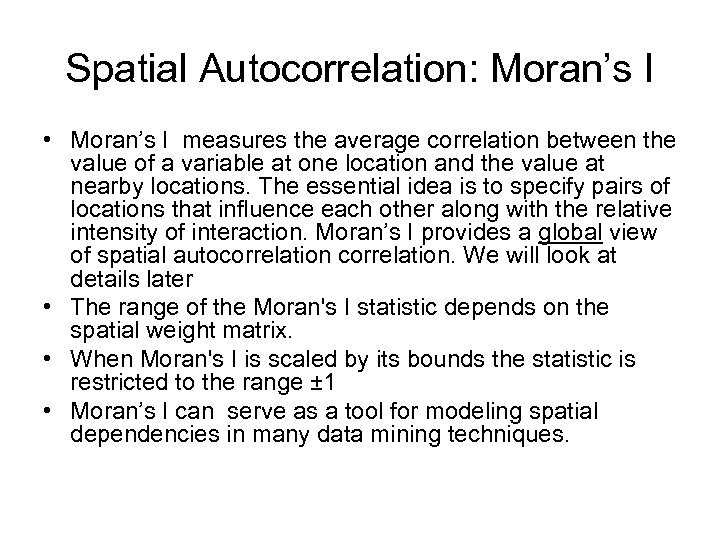Spatial Autocorrelation: Moran’s I • Moran’s I measures the average correlation between the value of a variable at one location and the value at nearby locations. The essential idea is to specify pairs of locations that influence each other along with the relative intensity of interaction. Moran’s I provides a global view of spatial autocorrelation. We will look at details later • The range of the Moran's I statistic depends on the spatial weight matrix. • When Moran's I is scaled by its bounds the statistic is restricted to the range ± 1 • Moran’s I can serve as a tool for modeling spatial dependencies in many data mining techniques.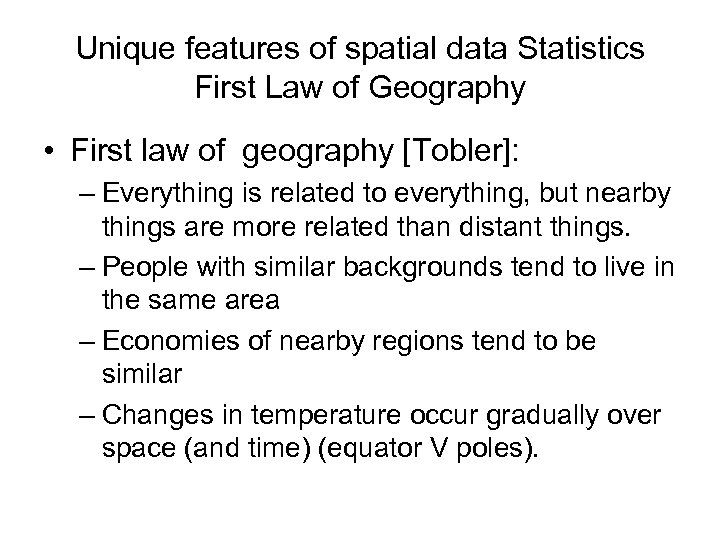Unique features of spatial data Statistics First Law of Geography • First law of geography [Tobler]: – Everything is related to everything, but nearby things are more related than distant things. – People with similar backgrounds tend to live in the same area – Economies of nearby regions tend to be similar – Changes in temperature occur gradually over space (and time) (equator V poles).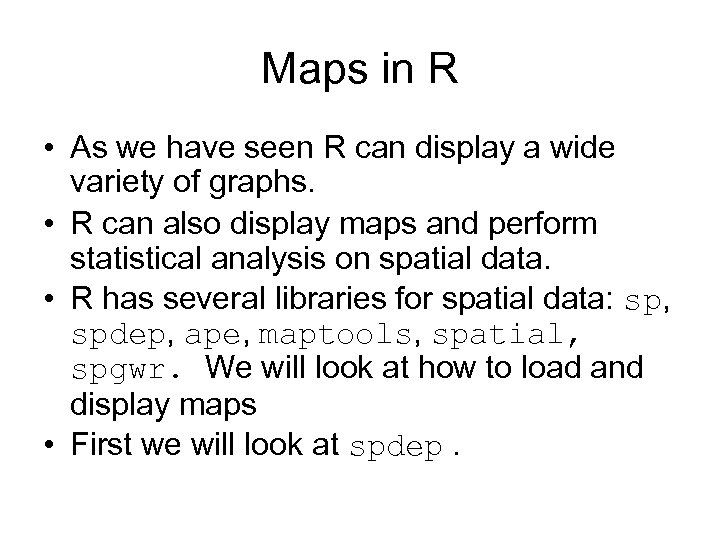Maps in R • As we have seen R can display a wide variety of graphs. • R can also display maps and perform statistical analysis on spatial data. • R has several libraries for spatial data: sp, spdep, ape, maptools, spatial, spgwr. We will look at how to load and display maps • First we will look at spdep.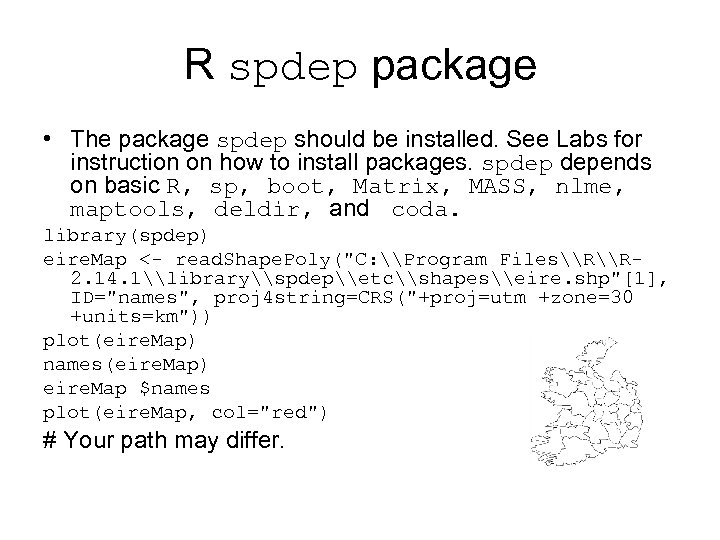R spdep package • The package spdep should be installed. See Labs for instruction on how to install packages. spdep depends on basic R, sp, boot, Matrix, MASS, nlme, maptools, deldir, and coda. library(spdep) eire. Map <- read. Shape. Poly("C: \Program Files\R\R 2. 14. 1\library\spdep\etc\shapes\eire. shp", ID="names", proj 4 string=CRS("+proj=utm +zone=30 +units=km")) plot(eire. Map) names(eire. Map) eire. Map \$names plot(eire. Map, col="red") # Your path may differ.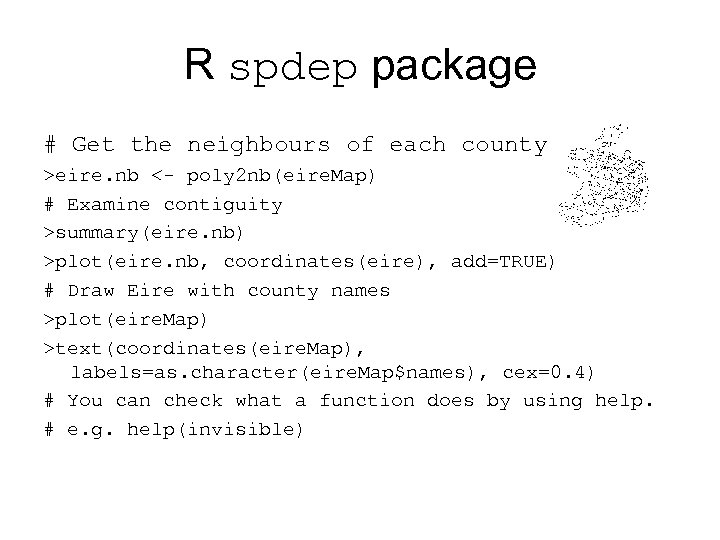R spdep package # Get the neighbours of each county. >eire. nb <- poly 2 nb(eire. Map) # Examine contiguity >summary(eire. nb) >plot(eire. nb, coordinates(eire), add=TRUE) # Draw Eire with county names >plot(eire. Map) >text(coordinates(eire. Map), labels=as. character(eire. Map\$names), cex=0. 4) # You can check what a function does by using help. # e. g. help(invisible)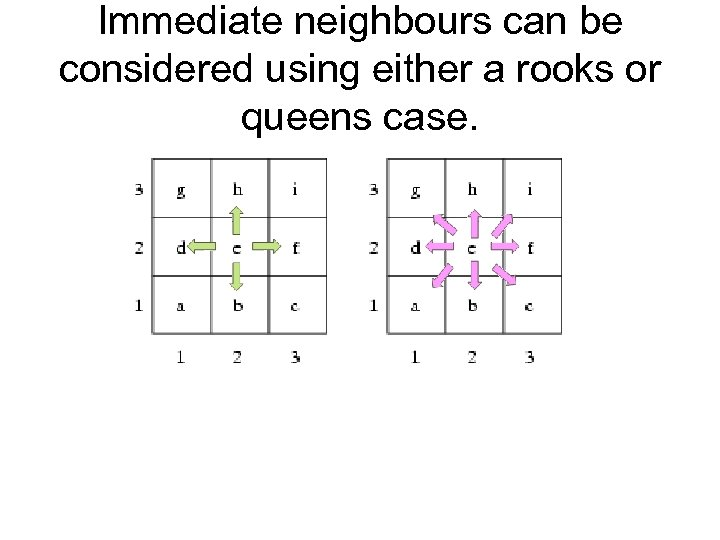Immediate neighbours can be considered using either a rooks or queens case.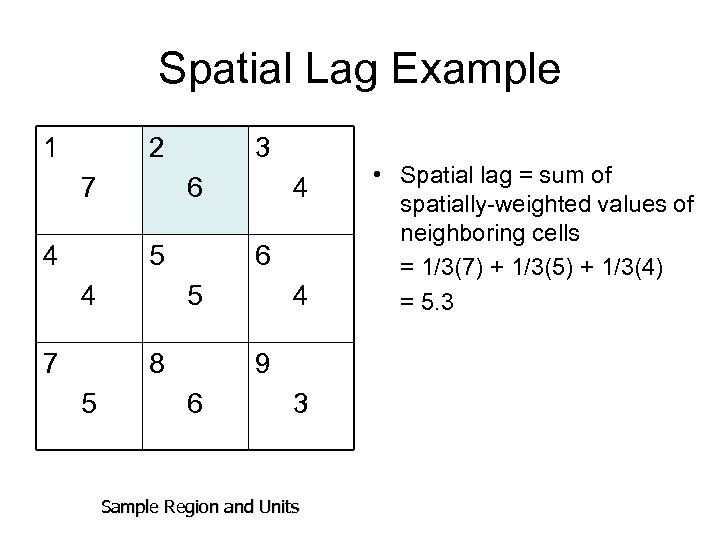Spatial Lag Example 1 2 7 4 3 6 5 4 7 6 5 8 5 4 4 9 6 3 Sample Region and Units • Spatial lag = sum of spatially-weighted values of neighboring cells = 1/3(7) + 1/3(5) + 1/3(4) = 5. 3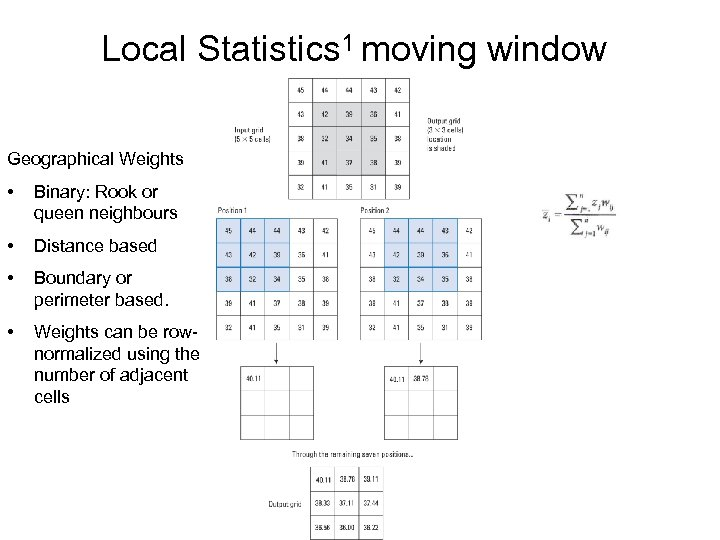Local Statistics 1 moving window Geographical Weights • Binary: Rook or queen neighbours • Distance based • Boundary or perimeter based. • Weights can be rownormalized using the number of adjacent cells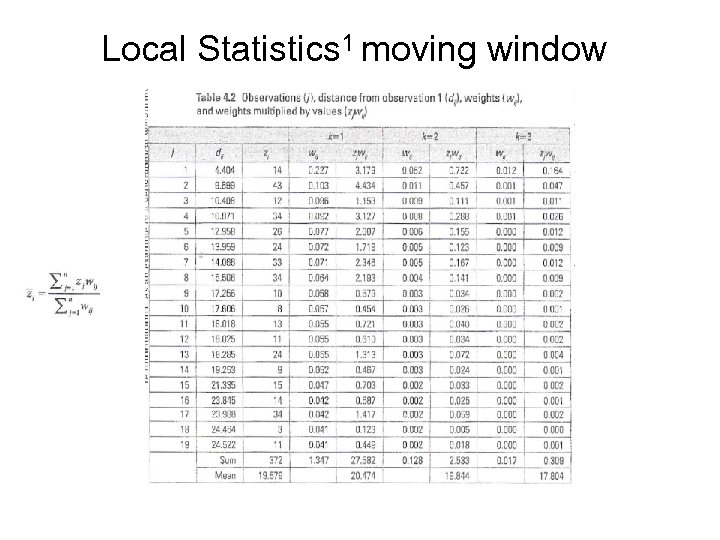Local Statistics 1 moving window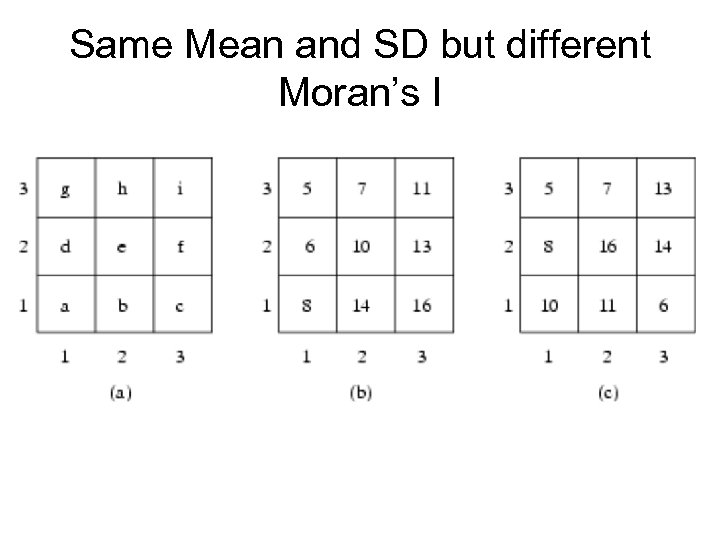Same Mean and SD but different Moran’s I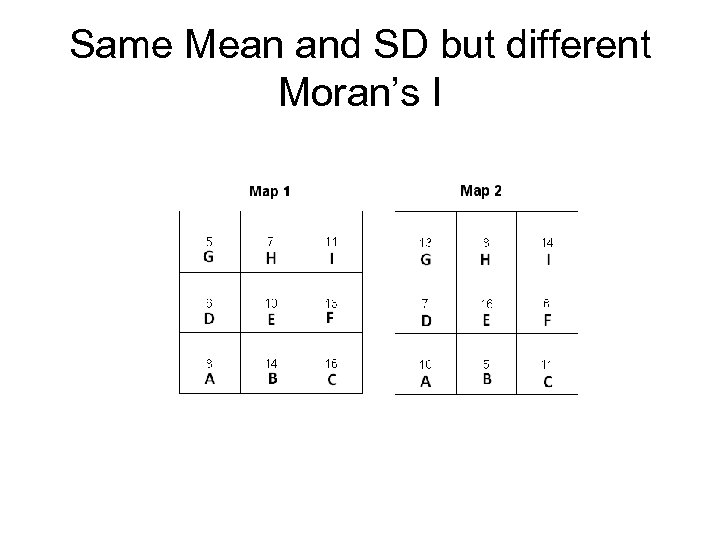Same Mean and SD but different Moran’s I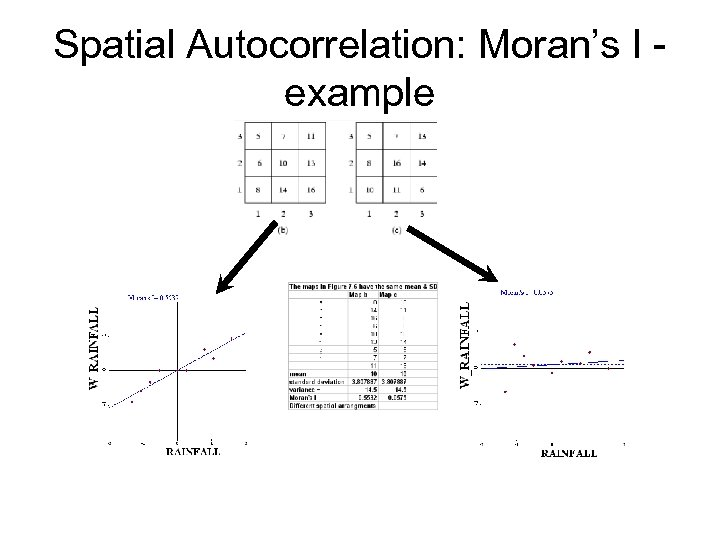Spatial Autocorrelation: Moran’s I example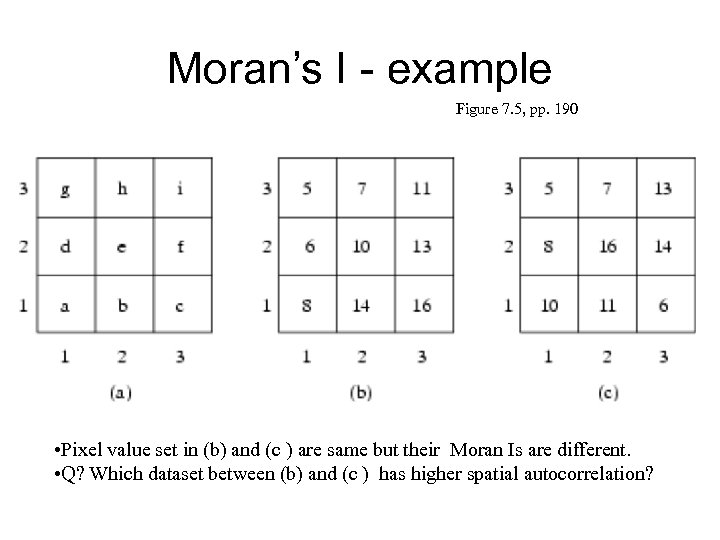Moran’s I - example Figure 7. 5, pp. 190 • Pixel value set in (b) and (c ) are same but their Moran Is are different. • Q? Which dataset between (b) and (c ) has higher spatial autocorrelation?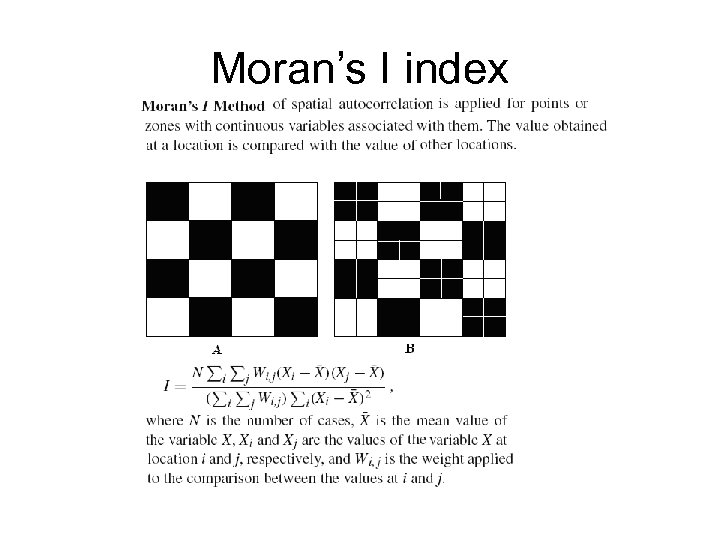Moran’s I index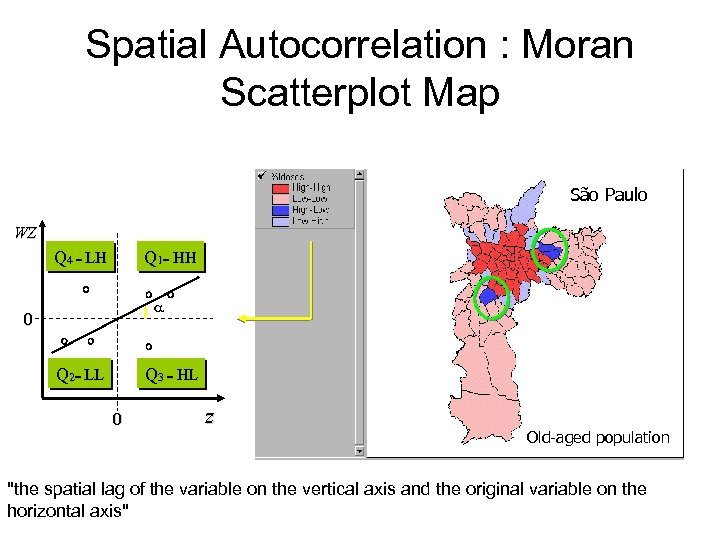Spatial Autocorrelation : Moran Scatterplot Map São Paulo WZ Q 4 = LH Q 1= HH a 0 Q 2= LL Q 3 = HL 0 z Old-aged population "the spatial lag of the variable on the vertical axis and the original variable on the horizontal axis"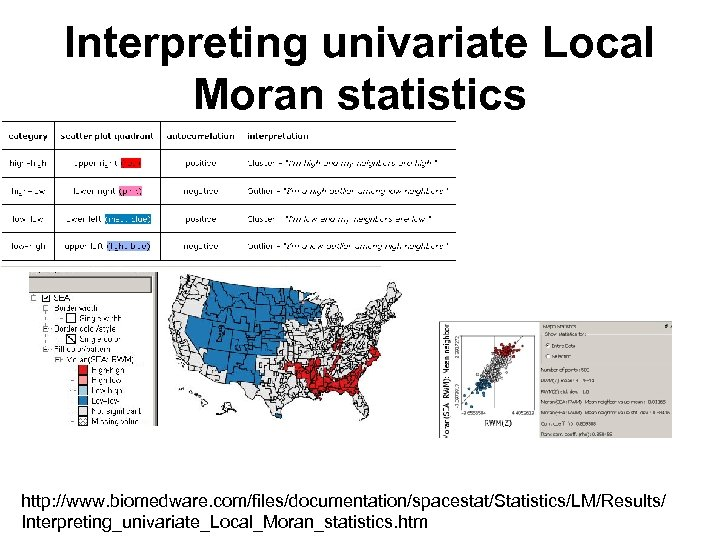Interpreting univariate Local Moran statistics http: //www. biomedware. com/files/documentation/spacestat/Statistics/LM/Results/ Interpreting_univariate_Local_Moran_statistics. htm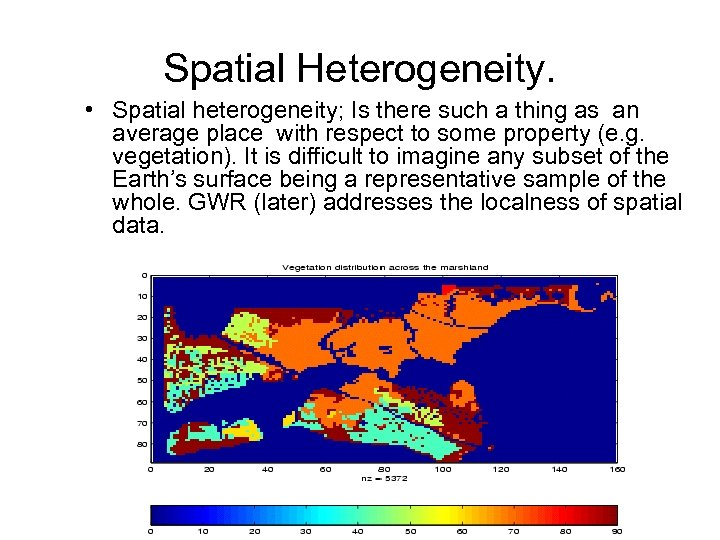Spatial Heterogeneity. • Spatial heterogeneity; Is there such a thing as an average place with respect to some property (e. g. vegetation). It is difficult to imagine any subset of the Earth’s surface being a representative sample of the whole. GWR (later) addresses the localness of spatial data.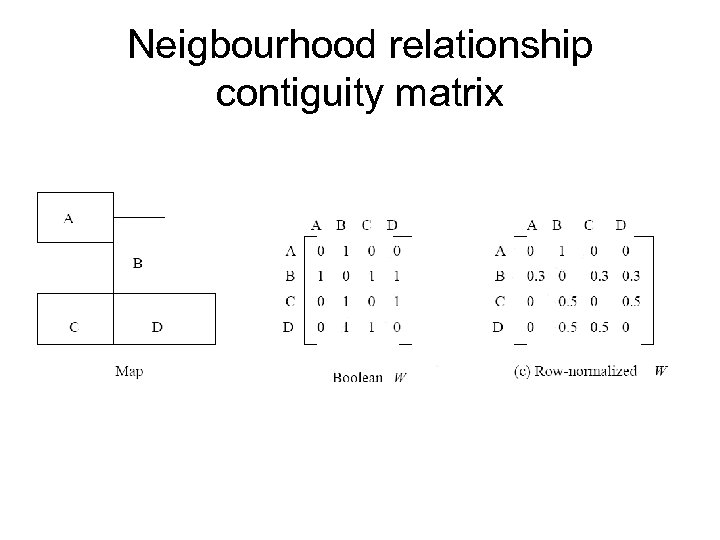Neigbourhood relationship contiguity matrix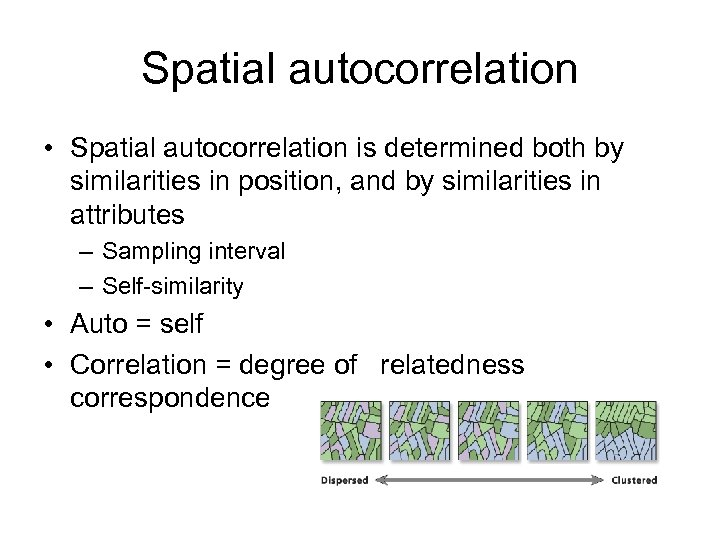Spatial autocorrelation • Spatial autocorrelation is determined both by similarities in position, and by similarities in attributes – Sampling interval – Self-similarity • Auto = self • Correlation = degree of relatedness correspondence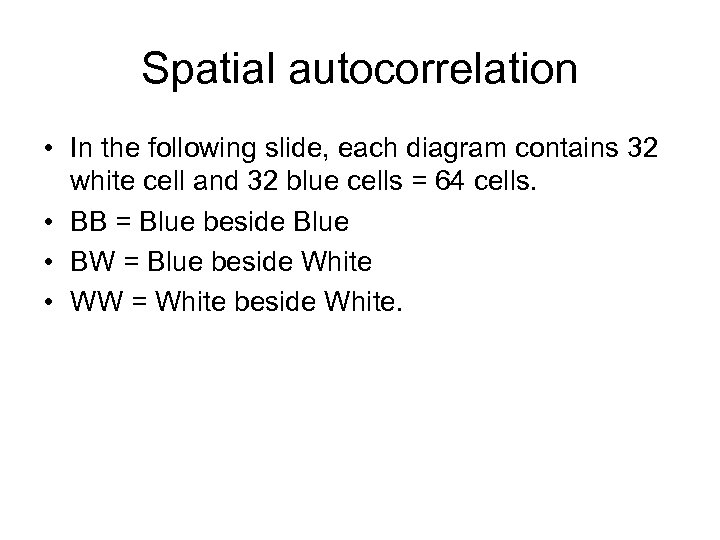Spatial autocorrelation • In the following slide, each diagram contains 32 white cell and 32 blue cells = 64 cells. • BB = Blue beside Blue • BW = Blue beside White • WW = White beside White.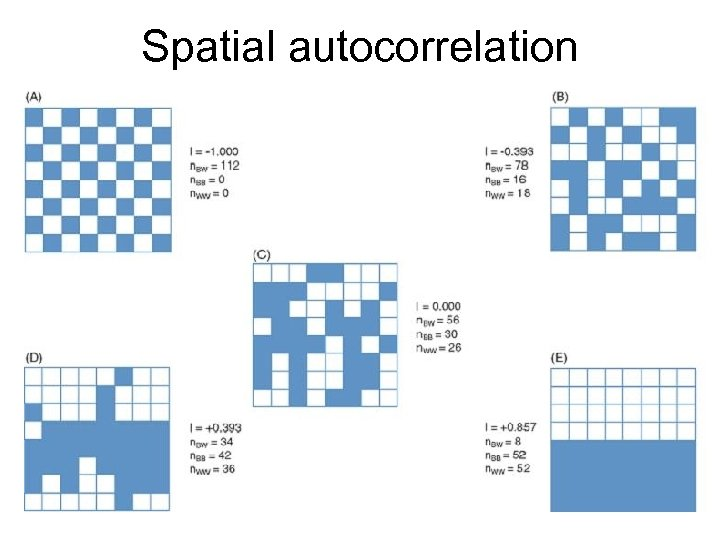Spatial autocorrelation Negative Dispersed Spatial Independence Spatial Clustering Positive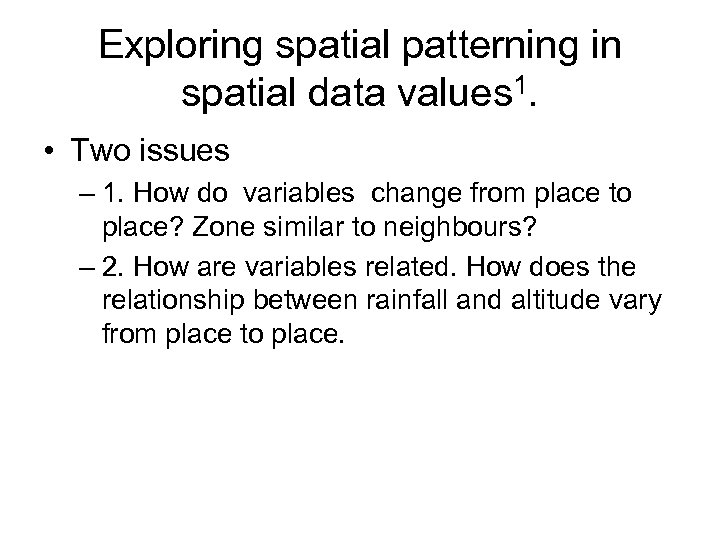Exploring spatial patterning in spatial data values 1. • Two issues – 1. How do variables change from place to place? Zone similar to neighbours? – 2. How are variables related. How does the relationship between rainfall and altitude vary from place to place.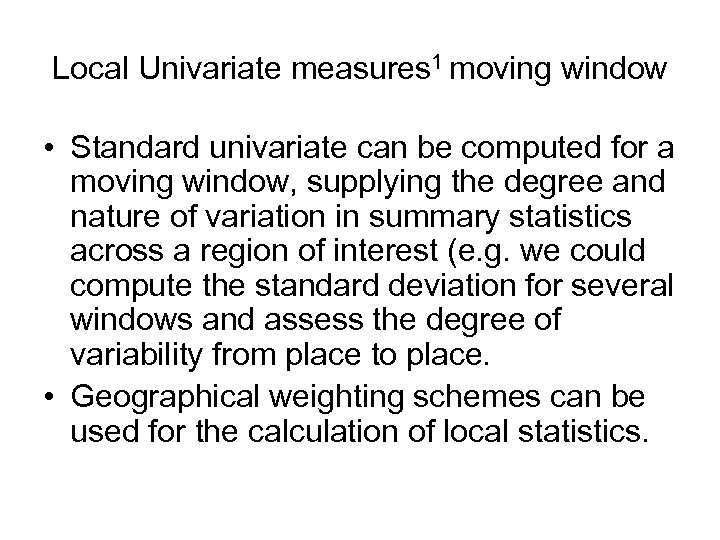Local Univariate measures 1 moving window • Standard univariate can be computed for a moving window, supplying the degree and nature of variation in summary statistics across a region of interest (e. g. we could compute the standard deviation for several windows and assess the degree of variability from place to place. • Geographical weighting schemes can be used for the calculation of local statistics.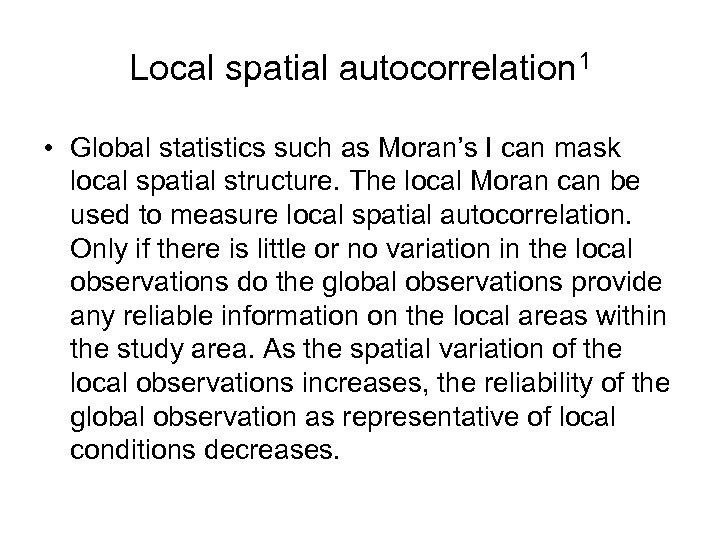Local spatial autocorrelation 1 • Global statistics such as Moran’s I can mask local spatial structure. The local Moran can be used to measure local spatial autocorrelation. Only if there is little or no variation in the local observations do the global observations provide any reliable information on the local areas within the study area. As the spatial variation of the local observations increases, the reliability of the global observation as representative of local conditions decreases.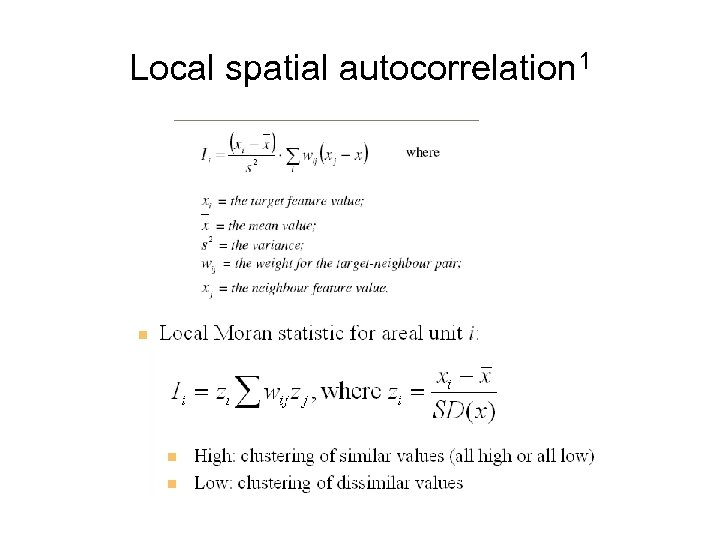Local spatial autocorrelation 1 The weights could be based on rook, queen, distance, perimeter and normalized by number of neighbours ( slide 28)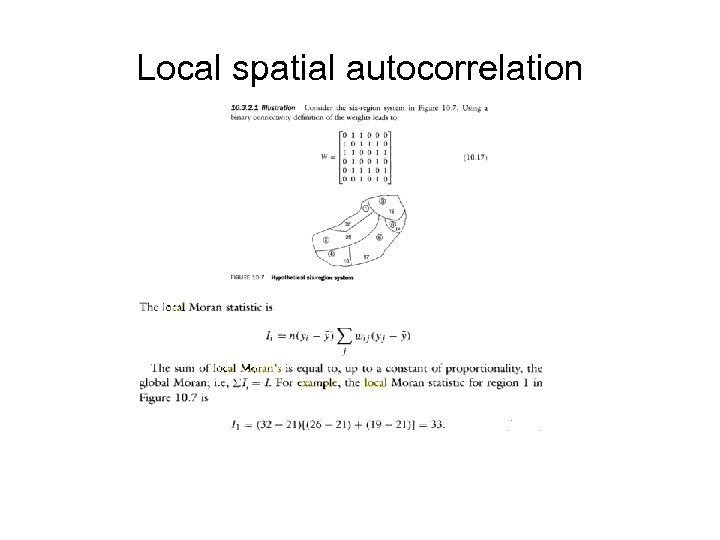Local spatial autocorrelation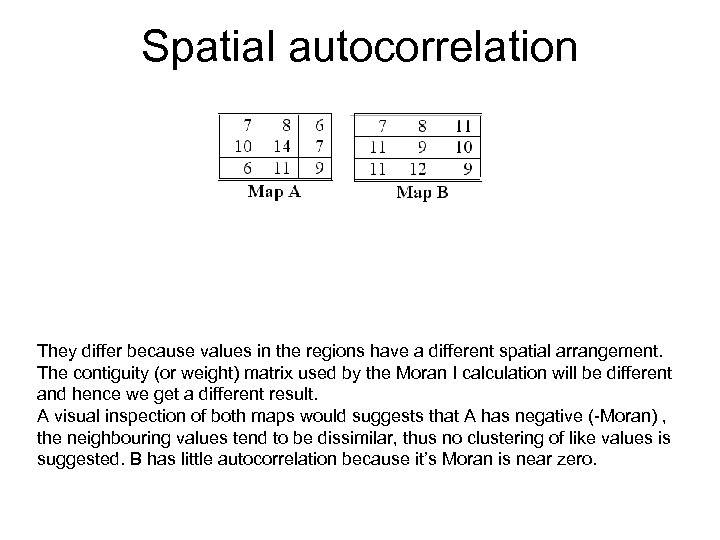Spatial autocorrelation Negative Dispersed Spatial Map A and Map B each represent a distinct geographic region. The number in the Independence regions (cells) represents the number of leukaemia cases in that region. These two sets of values have the same mean and standard deviation. In contrast, Moran’s I statistic for the data on Map A is -0. 269, and 0. 041 for the data on Map B. Positive Spatial because They differ. Clusteringvalues in the regions have a different spatial arrangement. The contiguity (or weight) matrix used by the Moran I calculation will be different and hence we get a different result. A visual inspection of both maps would suggests that A has negative (-Moran) , the neighbouring values tend to be dissimilar, thus no clustering of like values is suggested. B has little autocorrelation because it’s Moran is near zero.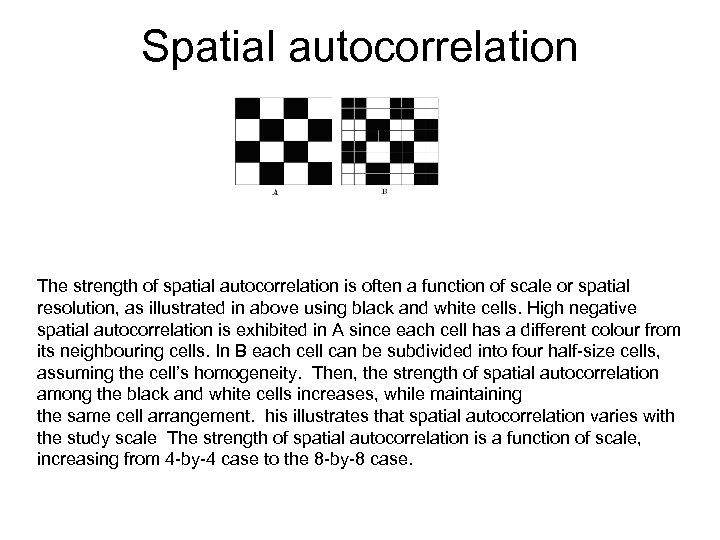Spatial autocorrelation Negative Dispersed Spatial The grids A and B represent two different spatial resolutions over the same area. Independence Grid A contains 16 cells and Grid B contains 64 cells. The strength of spatial autocorrelation is often a function of scale or spatial resolution, as illustrated in above using black and white cells. High negative spatial autocorrelation is exhibited in A since each cell has a different colour from Positive Spatial Clustering its neighbouring cells. In B each cell can be subdivided into four half-size cells, assuming the cell’s homogeneity. Then, the strength of spatial autocorrelation among the black and white cells increases, while maintaining the same cell arrangement. his illustrates that spatial autocorrelation varies with the study scale The strength of spatial autocorrelation is a function of scale, increasing from 4 -by-4 case to the 8 -by-8 case.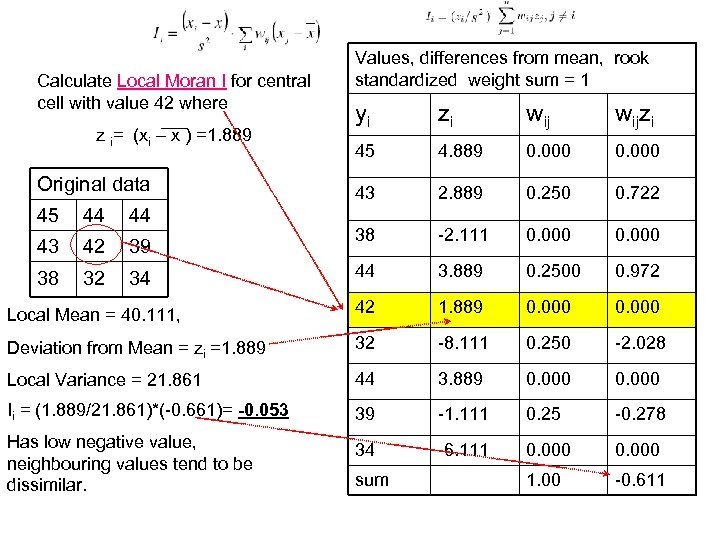Calculate Local Moran I for central cell with value 42 where Values, differences from mean, rook standardized weight sum = 1 yi zi wijzi 45 4. 889 0. 000 43 2. 889 0. 250 0. 722 38 -2. 111 0. 000 44 3. 889 0. 2500 0. 972 Local Mean = 40. 111, 42 1. 889 0. 000 Deviation from Mean = zi =1. 889 32 -8. 111 0. 250 -2. 028 Local Variance = 21. 861 44 3. 889 0. 000 Ii = (1. 889/21. 861)*(-0. 661)= -0. 053 39 -1. 111 0. 25 -0. 278 Has low negative value, neighbouring values tend to be dissimilar. 34 -6. 111 0. 000 1. 00 -0. 611 z i= (xi – x ) =1. 889 Original data 45 44 44 43 42 39 38 32 34 sum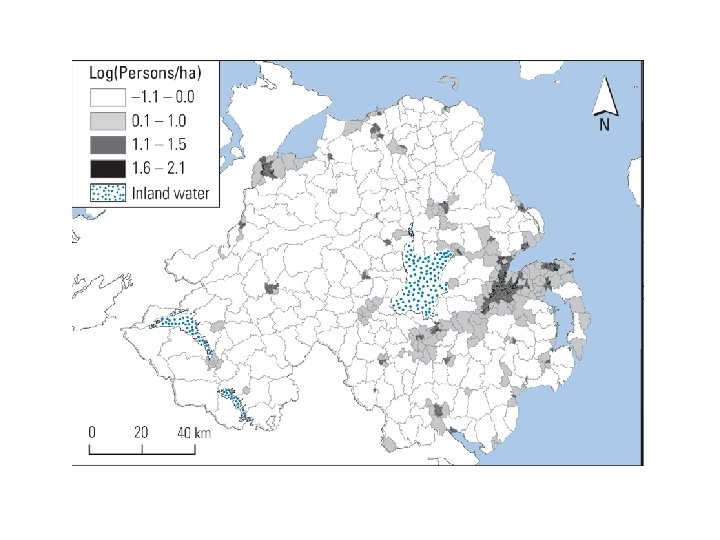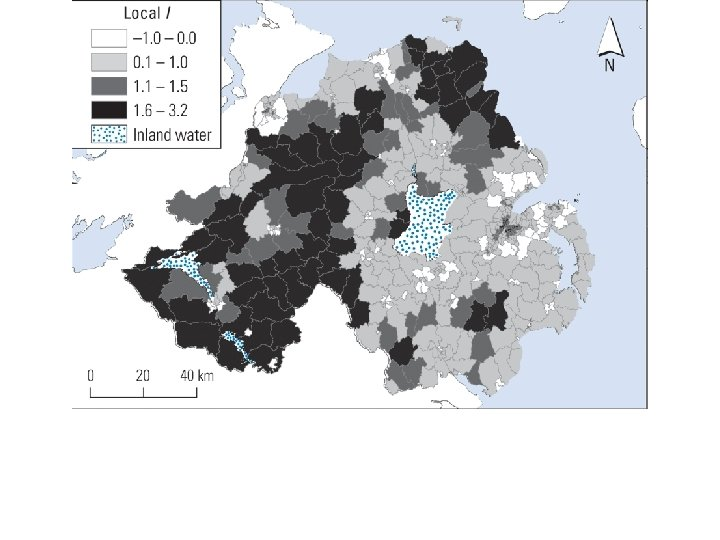Global Moran’s I = 0. 665 Local I, large positive values in rural areas, more patchy around Belfast Local I for log of persons per hectare in NI, 2001, queens contiguity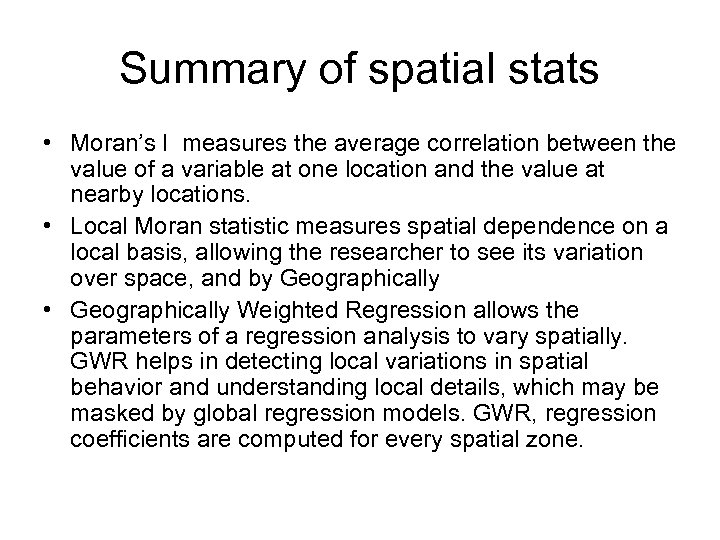Summary of spatial stats • Moran’s I measures the average correlation between the value of a variable at one location and the value at nearby locations. • Local Moran statistic measures spatial dependence on a local basis, allowing the researcher to see its variation over space, and by Geographically • Geographically Weighted Regression allows the parameters of a regression analysis to vary spatially. GWR helps in detecting local variations in spatial behavior and understanding local details, which may be masked by global regression models. GWR, regression coefficients are computed for every spatial zone.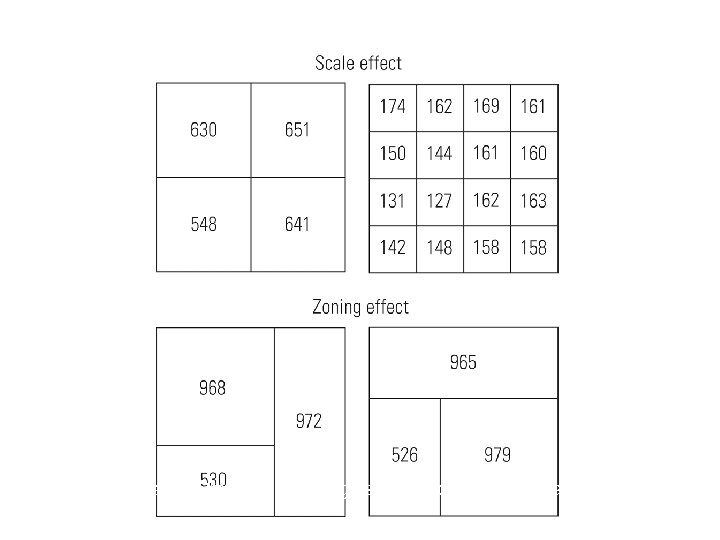© Oxford University Press, 2010. All rights reserved. Lloyd: Spatial Data Analysis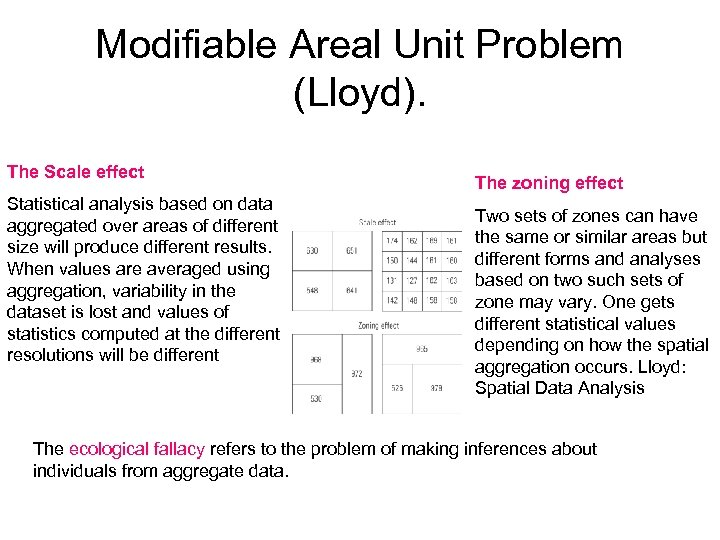Modifiable Areal Unit Problem (Lloyd). The Scale effect Statistical analysis based on data aggregated over areas of different size will produce different results. When values are averaged using aggregation, variability in the dataset is lost and values of statistics computed at the different resolutions will be different The zoning effect Two sets of zones can have the same or similar areas but different forms and analyses based on two such sets of zone may vary. One gets different statistical values depending on how the spatial aggregation occurs. Lloyd: Spatial Data Analysis The ecological fallacy refers to the problem of making inferences about individuals from aggregate data.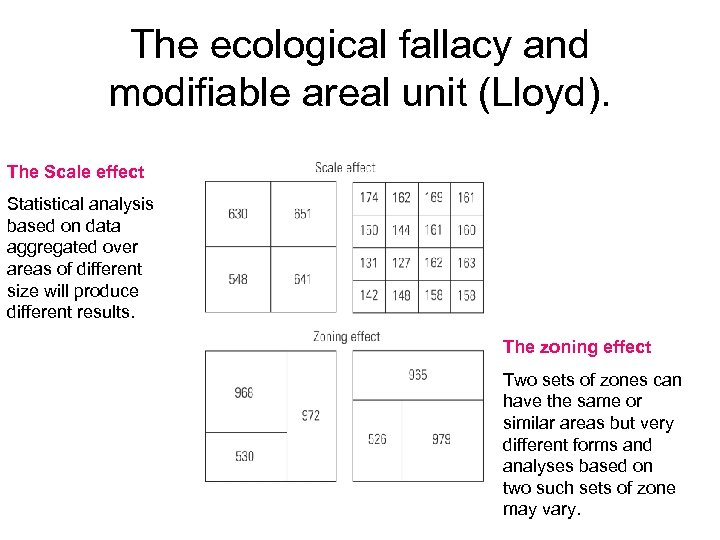The ecological fallacy and modifiable areal unit (Lloyd). The Scale effect Statistical analysis based on data aggregated over areas of different size will produce different results. The zoning effect Two sets of zones can have the same or similar areas but very different forms and analyses based on two such sets of zone may vary.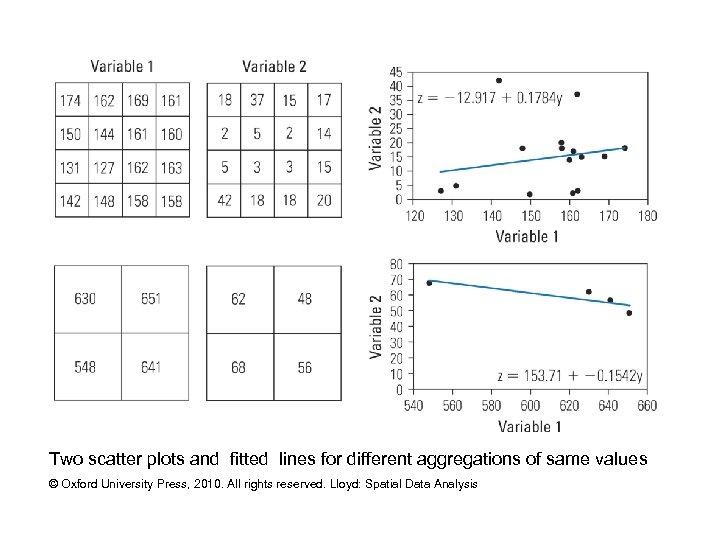Two scatter plots and fitted lines for different aggregations of same values © Oxford University Press, 2010. All rights reserved. Lloyd: Spatial Data Analysis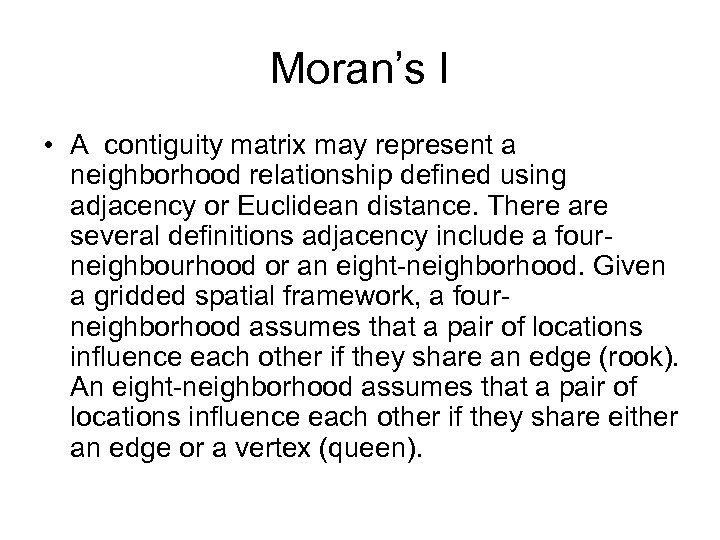Moran’s I • A contiguity matrix may represent a neighborhood relationship defined using adjacency or Euclidean distance. There are several definitions adjacency include a fourneighbourhood or an eight-neighborhood. Given a gridded spatial framework, a fourneighborhood assumes that a pair of locations influence each other if they share an edge (rook). An eight-neighborhood assumes that a pair of locations influence each other if they share either an edge or a vertex (queen).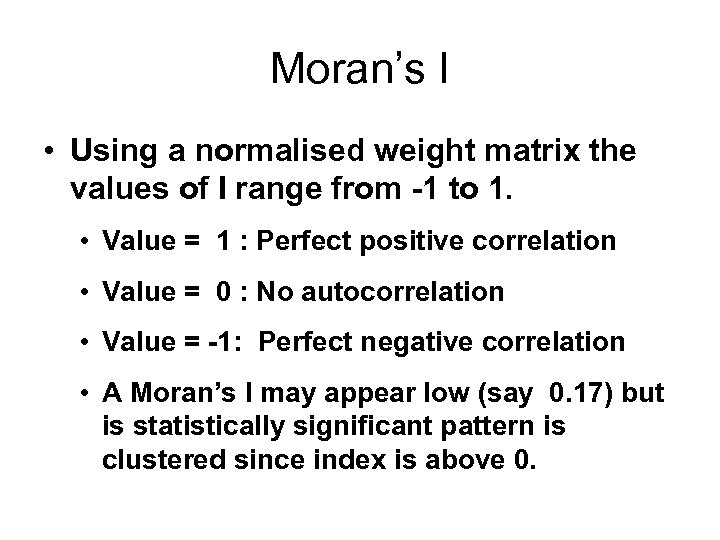Moran’s I • Using a normalised weight matrix the values of I range from -1 to 1. • Value = 1 : Perfect positive correlation • Value = 0 : No autocorrelation • Value = -1: Perfect negative correlation • A Moran’s I may appear low (say 0. 17) but is statistically significant pattern is clustered since index is above 0.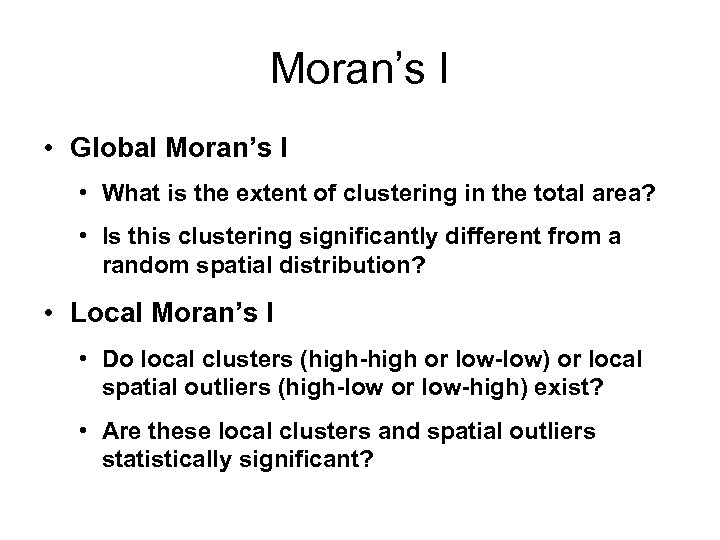Moran’s I • Global Moran’s I • What is the extent of clustering in the total area? • Is this clustering significantly different from a random spatial distribution? • Local Moran’s I • Do local clusters (high-high or low-low) or local spatial outliers (high-low or low-high) exist? • Are these local clusters and spatial outliers statistically significant?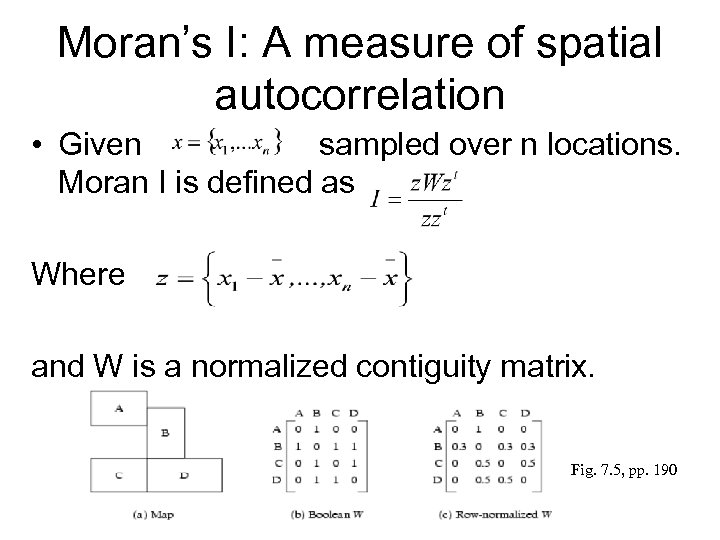Moran’s I: A measure of spatial autocorrelation • Given sampled over n locations. Moran I is defined as Where and W is a normalized contiguity matrix. Fig. 7. 5, pp. 190Spatial autocorrelation Negative Dispersed Spatial The grids A and B represent two different spatial resolutions over the same area. Independence Grid A contains 16 cells and Grid B contains 64 cells. The strength of spatial autocorrelation is often a function of scale or spatial resolution, as illustrated in above using black and white cells. High negative spatial autocorrelation is exhibited in A since each cell has a different colour from Positive Spatial Clustering its neighbouring cells. In B each cell can be subdivided into four half-size cells, assuming the cell’s homogeneity. Then, the strength of spatial autocorrelation among the black and white cells increases, while maintaining the same cell arrangement. his illustrates that spatial autocorrelation varies with the study scale The strength of spatial autocorrelation is a function of scale, increasing from 4 -by-4 case to the 8 -by-8 case.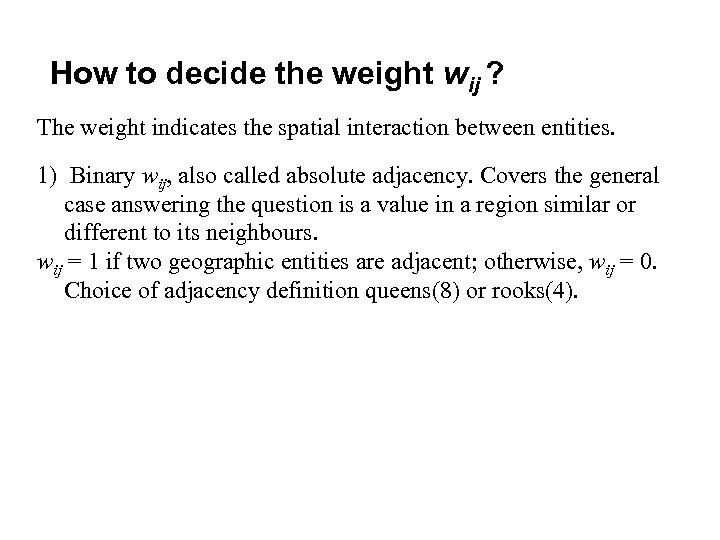How to decide the weight wij ? The weight indicates the spatial interaction between entities. 1) Binary wij, also called absolute adjacency. Covers the general case answering the question is a value in a region similar or different to its neighbours. wij = 1 if two geographic entities are adjacent; otherwise, wij = 0. Choice of adjacency definition queens(8) or rooks(4).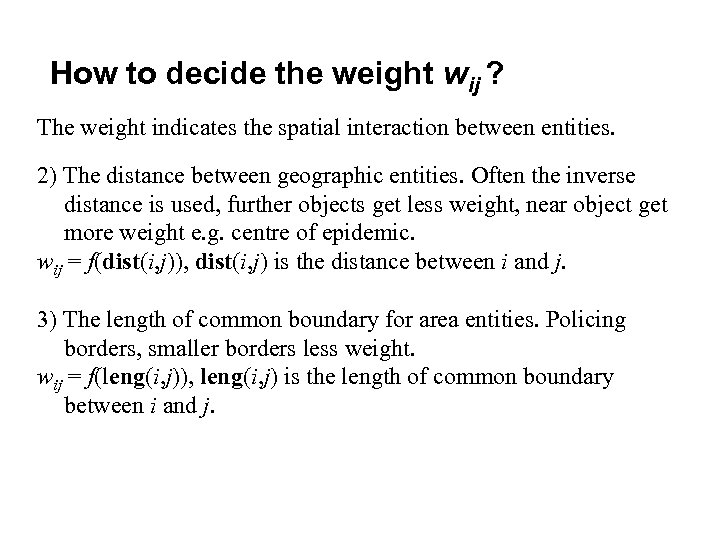How to decide the weight wij ? The weight indicates the spatial interaction between entities. 2) The distance between geographic entities. Often the inverse distance is used, further objects get less weight, near object get more weight e. g. centre of epidemic. wij = f(dist(i, j)), dist(i, j) is the distance between i and j. 3) The length of common boundary for area entities. Policing borders, smaller borders less weight. wij = f(leng(i, j)), leng(i, j) is the length of common boundary between i and j.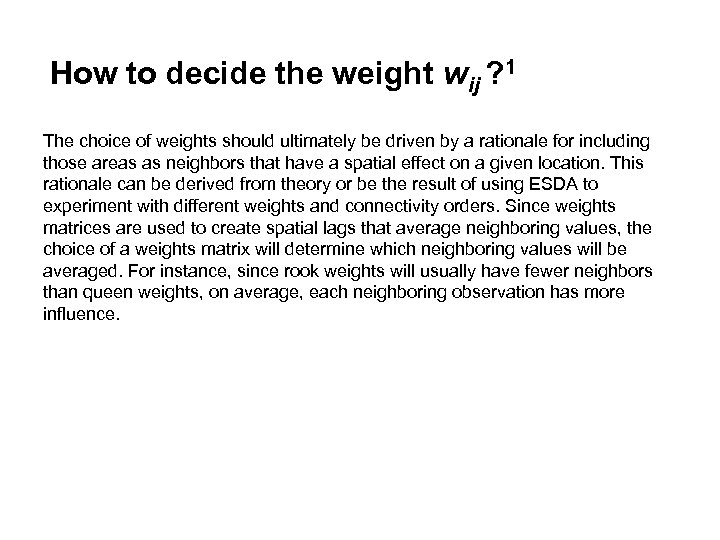How to decide the weight wij ? 1 The choice of weights should ultimately be driven by a rationale for including those areas as neighbors that have a spatial effect on a given location. This rationale can be derived from theory or be the result of using ESDA to experiment with different weights and connectivity orders. Since weights matrices are used to create spatial lags that average neighboring values, the choice of a weights matrix will determine which neighboring values will be averaged. For instance, since rook weights will usually have fewer neighbors than queen weights, on average, each neighboring observation has more influence.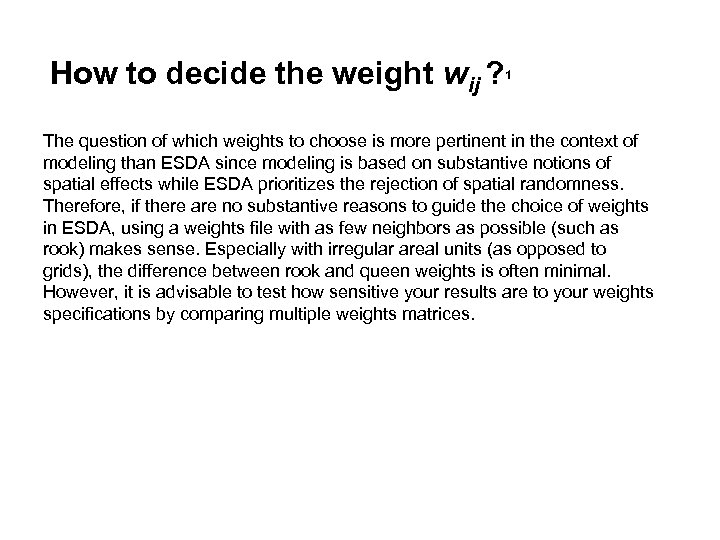How to decide the weight wij ? 1 The question of which weights to choose is more pertinent in the context of modeling than ESDA since modeling is based on substantive notions of spatial effects while ESDA prioritizes the rejection of spatial randomness. Therefore, if there are no substantive reasons to guide the choice of weights in ESDA, using a weights file with as few neighbors as possible (such as rook) makes sense. Especially with irregular areal units (as opposed to grids), the difference between rook and queen weights is often minimal. However, it is advisable to test how sensitive your results are to your weights specifications by comparing multiple weights matrices.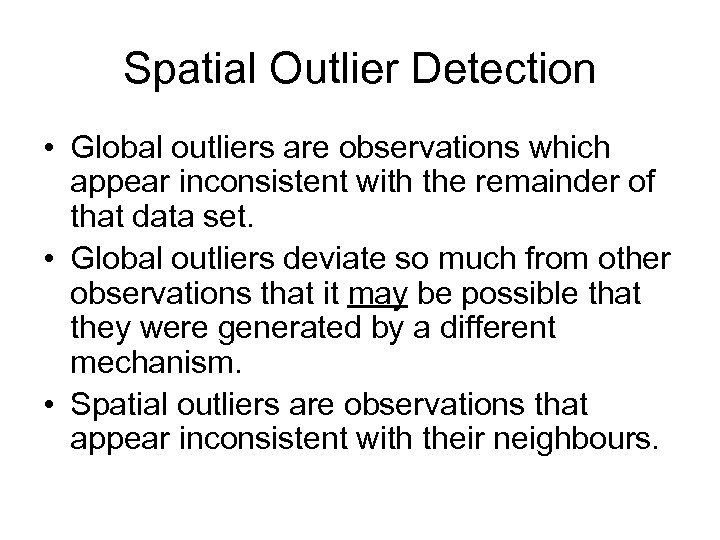Spatial Outlier Detection • Global outliers are observations which appear inconsistent with the remainder of that data set. • Global outliers deviate so much from other observations that it may be possible that they were generated by a different mechanism. • Spatial outliers are observations that appear inconsistent with their neighbours.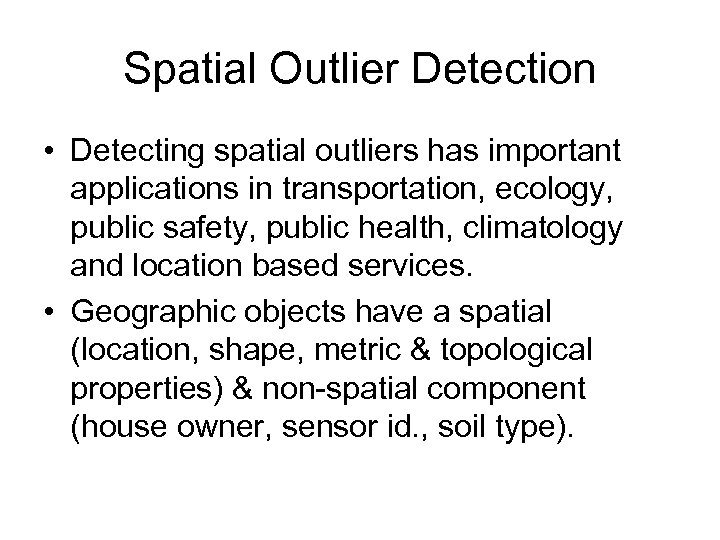Spatial Outlier Detection • Detecting spatial outliers has important applications in transportation, ecology, public safety, public health, climatology and location based services. • Geographic objects have a spatial (location, shape, metric & topological properties) & non-spatial component (house owner, sensor id. , soil type).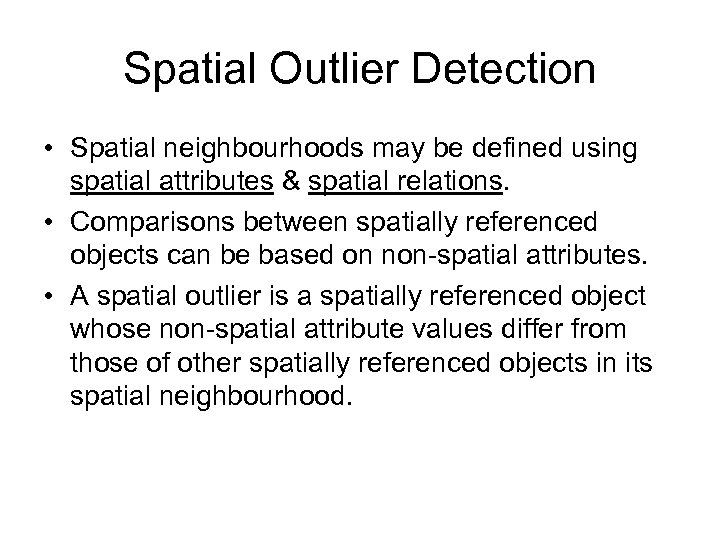Spatial Outlier Detection • Spatial neighbourhoods may be defined using spatial attributes & spatial relations. • Comparisons between spatially referenced objects can be based on non-spatial attributes. • A spatial outlier is a spatially referenced object whose non-spatial attribute values differ from those of other spatially referenced objects in its spatial neighbourhood.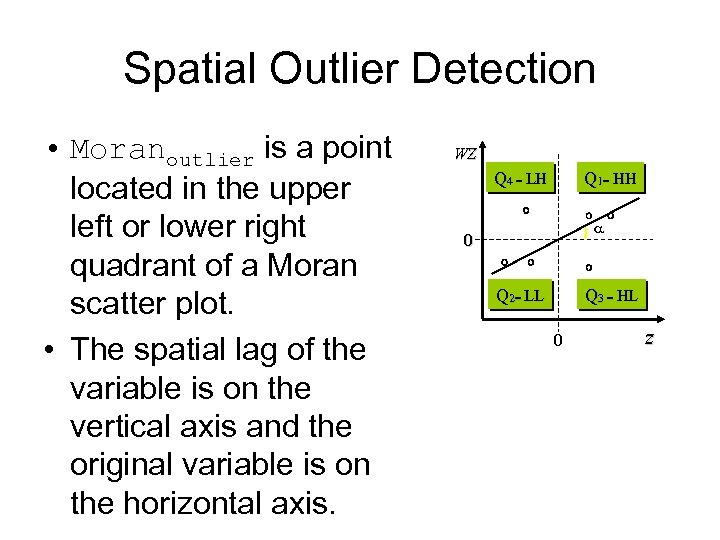Spatial Outlier Detection • Moranoutlier is a point located in the upper left or lower right quadrant of a Moran scatter plot. • The spatial lag of the variable is on the vertical axis and the original variable is on the horizontal axis. WZ Q 4 = LH Db 0 Q 2= LL Q 1= HH Cb a Q 3 = HL z 0 values in a given location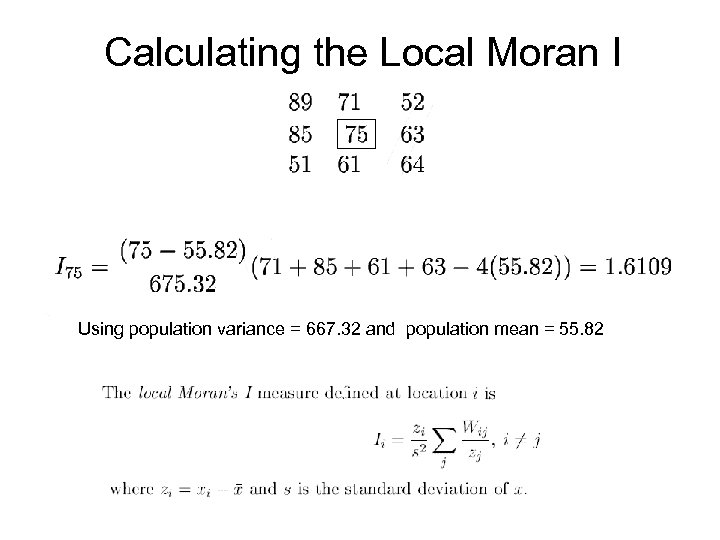Calculating the Local Moran I Using population variance = 667. 32 and population mean = 55. 82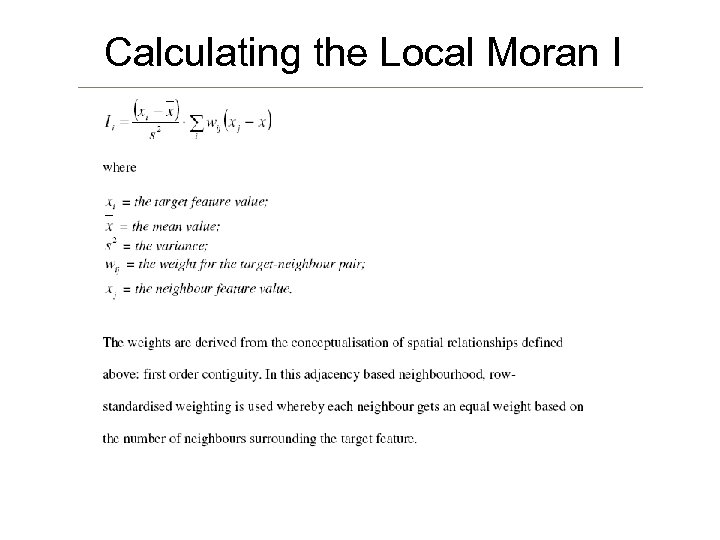Calculating the Local Moran I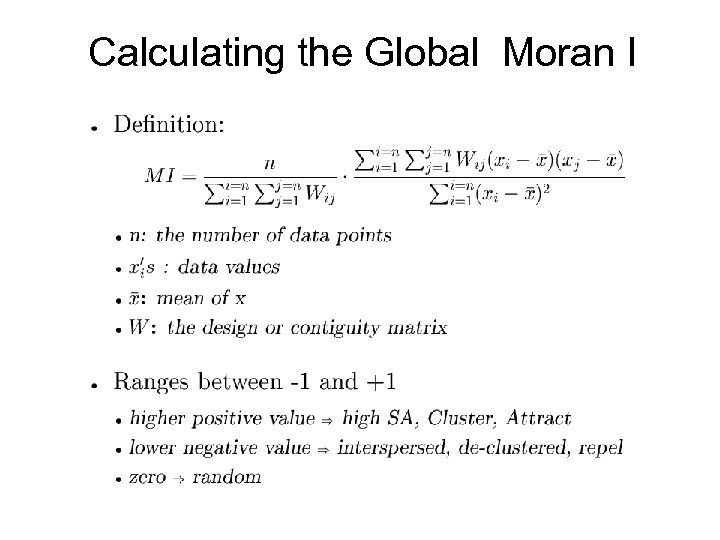Calculating the Global Moran I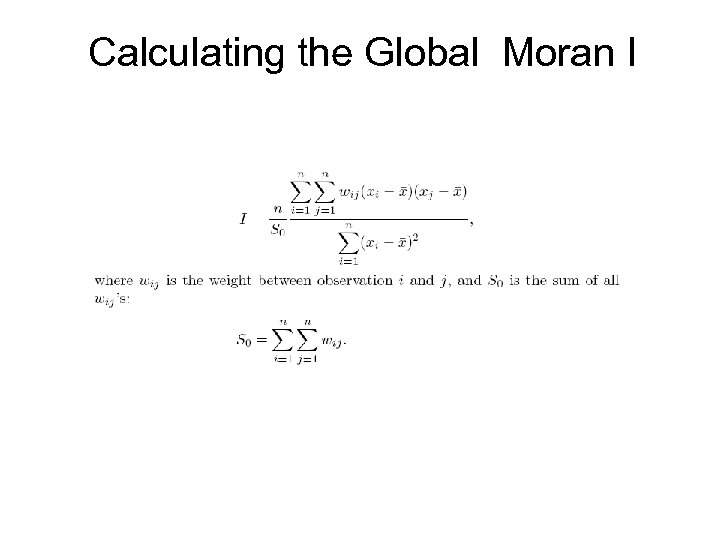Calculating the Global Moran I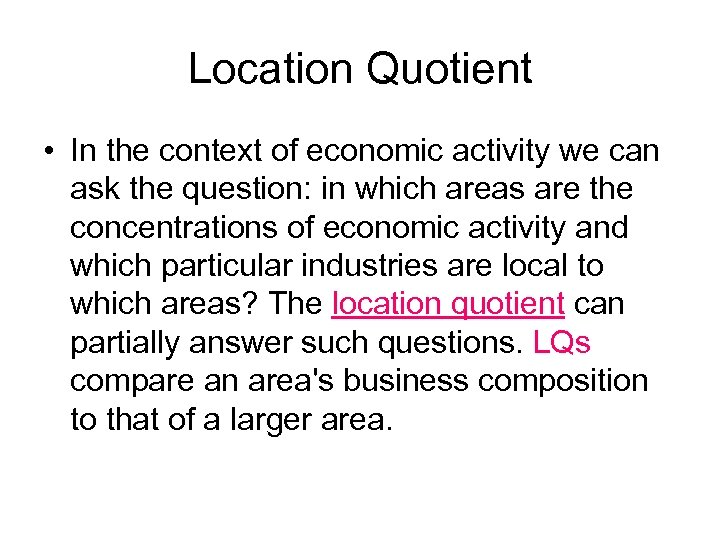Location Quotient • In the context of economic activity we can ask the question: in which areas are the concentrations of economic activity and which particular industries are local to which areas? The location quotient can partially answer such questions. LQs compare an area's business composition to that of a larger area.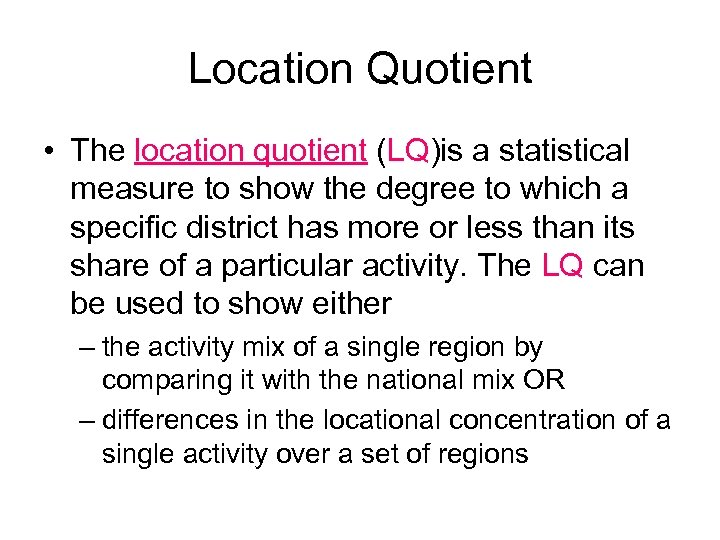Location Quotient • The location quotient (LQ)is a statistical measure to show the degree to which a specific district has more or less than its share of a particular activity. The LQ can be used to show either – the activity mix of a single region by comparing it with the national mix OR – differences in the locational concentration of a single activity over a set of regions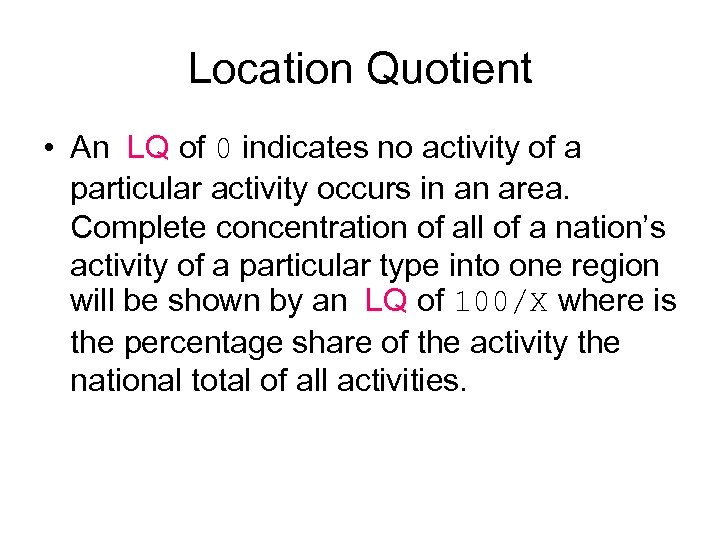Location Quotient • An LQ of 0 indicates no activity of a particular activity occurs in an area. Complete concentration of all of a nation’s activity of a particular type into one region will be shown by an LQ of 100/X where is the percentage share of the activity the national total of all activities.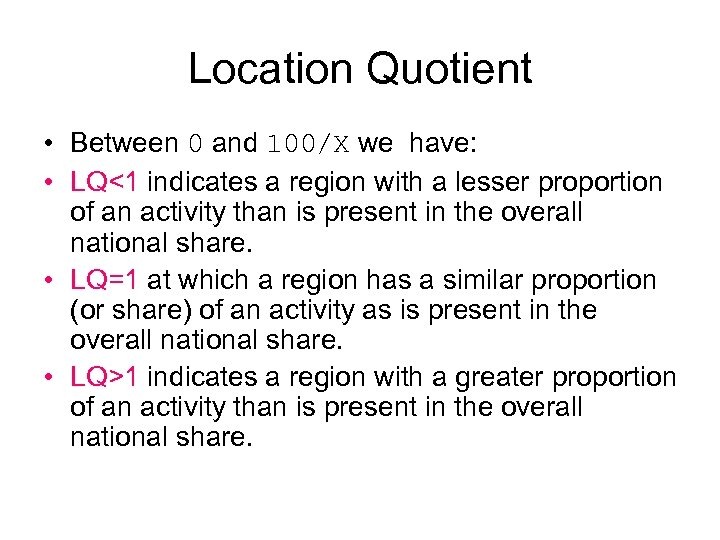Location Quotient • Between 0 and 100/X we have: • LQ<1 indicates a region with a lesser proportion of an activity than is present in the overall national share. • LQ=1 at which a region has a similar proportion (or share) of an activity as is present in the overall national share. • LQ>1 indicates a region with a greater proportion of an activity than is present in the overall national share.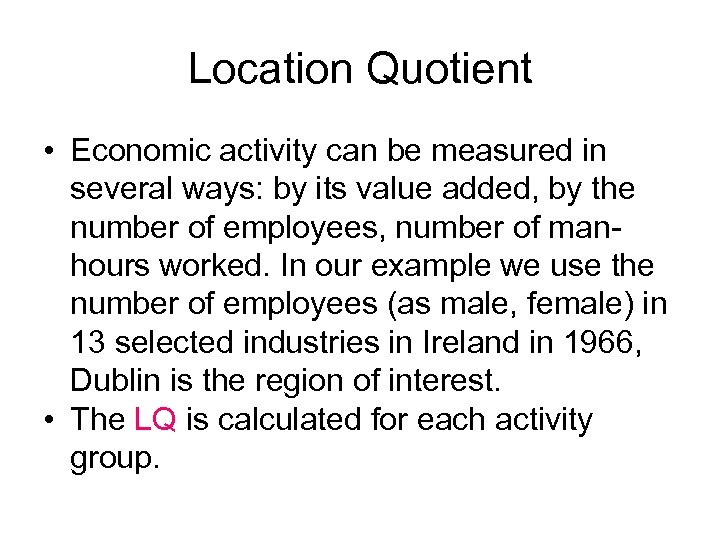Location Quotient • Economic activity can be measured in several ways: by its value added, by the number of employees, number of manhours worked. In our example we use the number of employees (as male, female) in 13 selected industries in Ireland in 1966, Dublin is the region of interest. • The LQ is calculated for each activity group.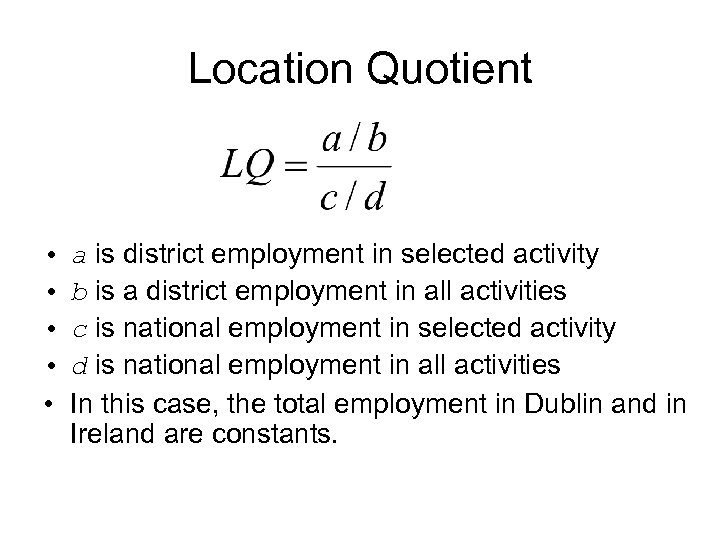Location Quotient • • • a is district employment in selected activity b is a district employment in all activities c is national employment in selected activity d is national employment in all activities In this case, the total employment in Dublin and in Ireland are constants.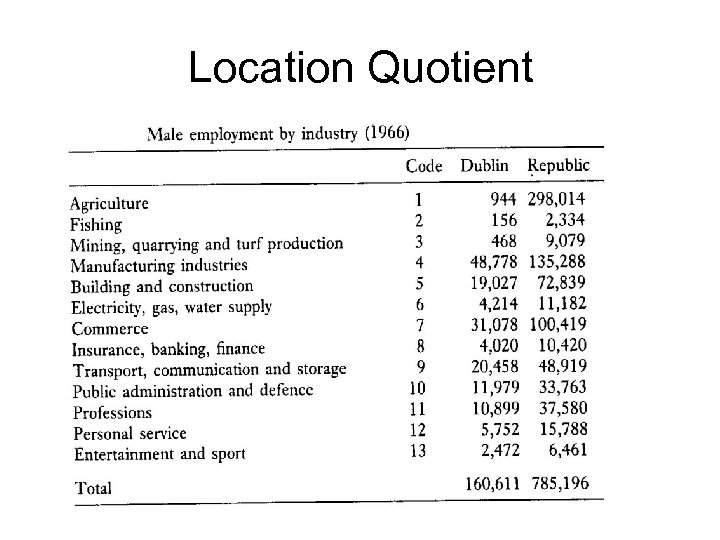Location Quotient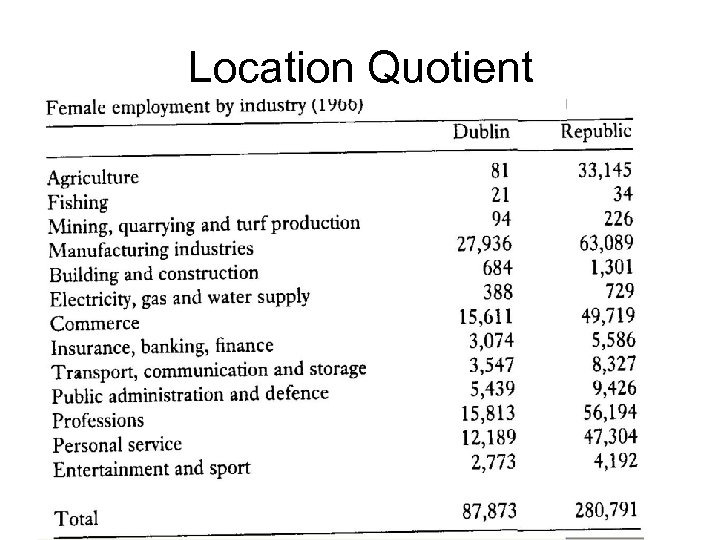Location Quotient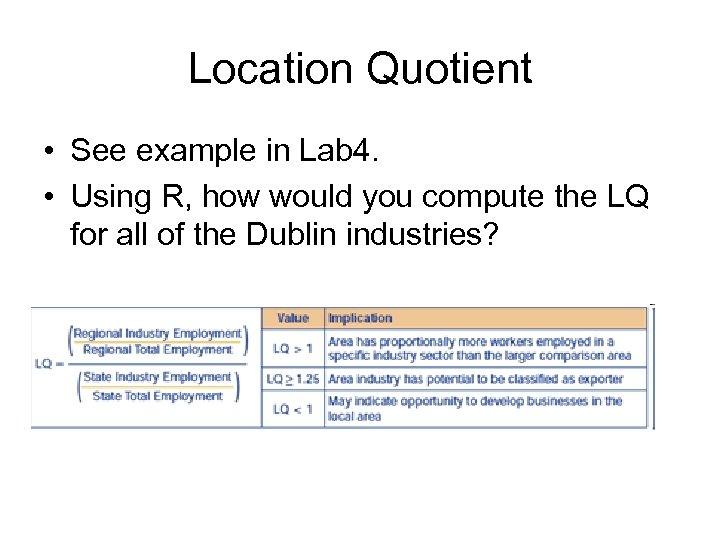Location Quotient • See example in Lab 4. • Using R, how would you compute the LQ for all of the Dublin industries?